24.1.16. Vector geometry¶

Computes geometric properties of the features in a vector layer and includes them in the output layer.

It generates a new vector layer with the same content as the input one, but with additional attributes, containing geometric measurements based on a selected CRS.

The attributes added to the table depend on the geometry type and dimension of the input layer:

• for point layers: X (xcoord), Y (ycoord), Z (zcoord) coordinates and/or M value (mvalue)

• for line layers: length and, for the LineString and CompoundCurve geometry types, the feature sinuosity and straight distance (straightdis)

• for polygon layers: perimeter and area

Default menu: Vector ► Geometry Tools

Parameters¶

Label

Имя

Тип

Описание

Input layer

INPUT

[vector: any]

Input vector layer

Calculate using

CALC_METHOD

[enumeration]

Default: 0

Calculation parameters to use for the geometric properties. One of:

• 0 — Layer CRS

• 1 — Project CRS

• 2 — Ellipsoidal

OUTPUT

[same as input]

Default: [Create temporary layer]

Specify the output (input copy with geometry) layer. One of:

• Create Temporary Layer (TEMPORARY_OUTPUT)

• Save to File…

• Save to Geopackage…

• Save to PostGIS Table…

The file encoding can also be changed here.

Outputs¶

Label

Имя

Тип

Описание

OUTPUT

[same as input]

Copy of the input vector layer with the addition of the geometry fields

Python code¶

import processing
processing.run("algorithm_id", {parameter_dictionary})

The algorithm id is displayed when you hover over the algorithm in the Processing Toolbox. The parameter dictionary provides the parameter NAMEs and values. See Изпользование алгоритмов геообработки в консоли for details on how to run processing algorithms from the Python console.

24.1.16.2. Affine transform¶

Applies an affine transformation to the layer geometries. Affine transformations can include translation, scaling and rotation. The operations are performed in the following order: scale, rotation, and translation.

Z and M values (if present) can be translated and scaled.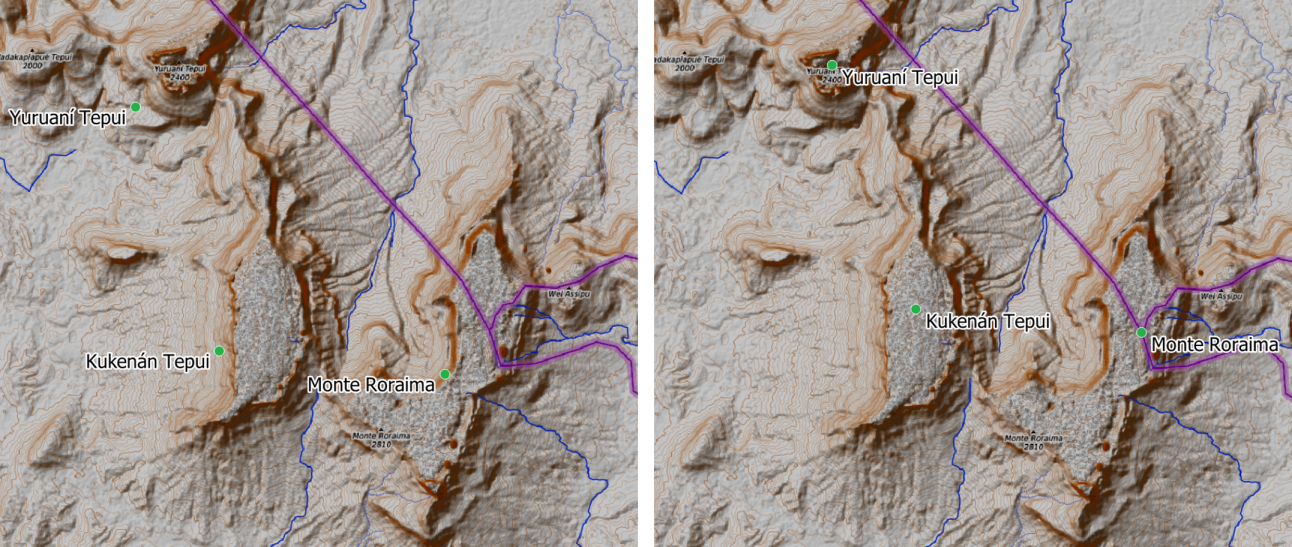Рис. 24.40 Vector point layer (green dots) before (left), and after (rigth) an affine transformation (translation).

См.также

Translate

Parameters¶

Label

Имя

Тип

Описание

Input layer

INPUT

[vector: any]

Input vector layer

Translation (x-axis)

DELTA_X

Default: 0

Displacement to apply on the X axis.

Translation (y-axis)

DELTA_Y

Default: 0

Displacement to apply on the Y axis.

Translation (z-axis)

DELTA_Z

Default: 0

Displacement to apply on the Z axis.

Translation (m-values)

DELTA_M

Default: 0

Offset to apply on m values.

Scale factor (x-axis)

SCALE_X

Default: 1

Scaling value (expansion or contraction) to apply on the X axis.

Scale factor (y-axis)

SCALE_Y

Default: 1

Scaling value (expansion or contraction) to apply on the Y axis.

Scale factor (z-axis)

SCALE_Z

Default: 1

Scaling value (expansion or contraction) to apply on the Z axis.

Scale factor (m-values)

SCALE_M

Default: 1

Scaling value (expansion or contraction) to apply on m values.

Rotation around z-axis (degrees counter-clockwise)

ROTATION_Z

Default: 0

Angle of the rotation in degrees.

Transformed

OUTPUT

[same as input]

Default: [Create temporary layer]

Specify the output vector layer. One of:

• Create Temporary Layer (TEMPORARY_OUTPUT)

• Save to File…

• Save to Geopackage…

• Save to PostGIS Table…

The file encoding can also be changed here.

Outputs¶

Label

Имя

Тип

Описание

Transformed

OUTPUT

[same as input]

Output (transformed) vector layer.

Python code¶

Algorithm ID: native:affinetransform

import processing
processing.run("algorithm_id", {parameter_dictionary})

The algorithm id is displayed when you hover over the algorithm in the Processing Toolbox. The parameter dictionary provides the parameter NAMEs and values. See Изпользование алгоритмов геообработки в консоли for details on how to run processing algorithms from the Python console.

24.1.16.3. Aggregate¶

Takes a vector or table layer and creates a new layer by aggregating features based on a group by expression.

Features for which group by expression returns the same value are grouped together.

It is possible to group all source features together using constant value in group by parameter, example: NULL.

It is also possible to group features by multiple fields using Array function, example: Array(«Field1», «Field2»).

Geometries (if present) are combined into one multipart geometry for each group. Output attributes are computed depending on each given aggregate definition.

This algorithm allows to use the default aggregates functions of the QGIS Expression engine.

См.также

Parameters¶

Label

Имя

Тип

Описание

Input layer

INPUT

[vector: any]

Input vector layer

Group by expression

GROUP_BY

[tablefield: any]

Default: „NULL“

Choose the grouping field. If NULL all features will be grouped.

Aggregates

AGGREGATES

[list]

List of output layer field definitions. Example of a field definition:

{„aggregate“: „sum“, „delimiter“: „,“, „input“: „ \$area“, „length“: 10, „name“: „totarea“, „precision“: 0, „type“: 6}

By default, the list contains all the fields of the input layer. In the GUI, you can edit these fields and their definitions, and you can also:

For each of the fields you’d like to retrieve information from, you need to define the following:

Input expression [expression] (input)

Field or expression from the input layer.

Aggregate function [enumeration] (aggregate)

Function to use on the input expression to return the aggregated value.

Default: concatenate (for string data type), sum (for numeric data type)

Delimiter [string] (delimiter)

Text string to separate aggregated values, for example in case of concatenation.

Default: ,

Output field name [string] (name)

Name of the aggregated field in the output layer. By default input field name is kept.

Type [enumeration] (type)

Data type of the output field. One of:

• 1 — Boolean

• 2 — Integer

• 4 — Integer64

• 6 — Double

• 10 — String

• 14 — Date

• 16 — DateTime

Length [number] (length)

Length of the output field.

Precision [number] (precision)

Precision of the output field.

GUI only

[vector: any]

You can load fields from another layer and use them for the aggregation

Aggregated

OUTPUT

[same as input]

Default: [Create temporary layer]

Specify the output (aggregate) layer One of:

• Create Temporary Layer (TEMPORARY_OUTPUT)

• Save to File…

• Save to Geopackage…

• Save to PostGIS Table…

The file encoding can also be changed here.

Outputs¶

Label

Имя

Тип

Описание

Aggregated

OUTPUT

[same as input]

Multigeometry vector layer with the aggregated values

Python code¶

Algorithm ID: native:aggregate

import processing
processing.run("algorithm_id", {parameter_dictionary})

The algorithm id is displayed when you hover over the algorithm in the Processing Toolbox. The parameter dictionary provides the parameter NAMEs and values. See Изпользование алгоритмов геообработки в консоли for details on how to run processing algorithms from the Python console.

24.1.16.4. Boundary¶

Returns the closure of the combinatorial boundary of the input geometries (i.e. the topological boundary of the geometry).

Only for polygon and line layers.

For polygon geometries , the boundary consists of all the lines making up the rings of the polygon.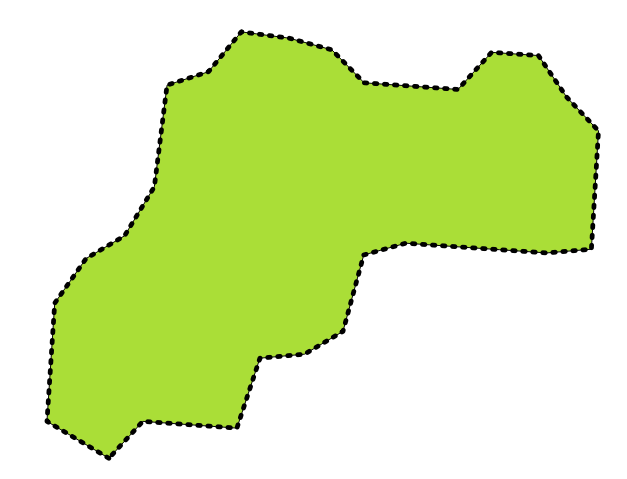Рис. 24.41 Boundaries (black dashed line) of the source polygon layer

For lines geometries, the boundaries are their end points.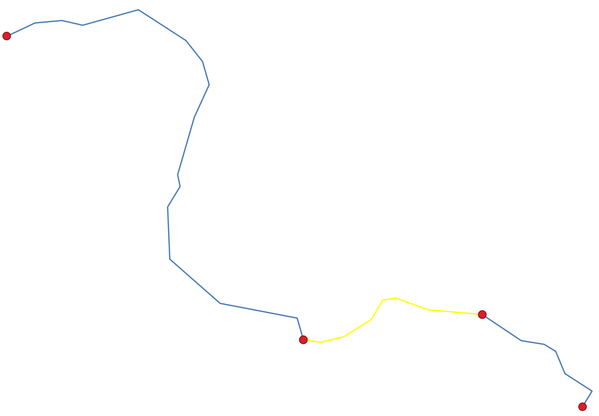Рис. 24.42 Boundary layer (red points) for lines. In yellow a selected feature.

Parameters¶

Label

Имя

Тип

Описание

Input layer

INPUT

[vector: line, polygon]

Input line or polygon vector layer

Boundary

OUTPUT

[vector: point, line]

Default: [Create temporary layer]

Specify the output (boundary) layer. One of:

• Create Temporary Layer (TEMPORARY_OUTPUT)

• Save to File…

• Save to Geopackage…

• Save to PostGIS Table…

The file encoding can also be changed here.

Outputs¶

Label

Имя

Тип

Описание

Boundary

OUTPUT

[vector: point, line]

Boundaries from the input layer (point for line, and line for polygon)

Python code¶

Algorithm ID: native:boundary

import processing
processing.run("algorithm_id", {parameter_dictionary})

The algorithm id is displayed when you hover over the algorithm in the Processing Toolbox. The parameter dictionary provides the parameter NAMEs and values. See Изпользование алгоритмов геообработки в консоли for details on how to run processing algorithms from the Python console.

24.1.16.5. Bounding boxes¶

Calculates the bounding box (envelope) of each feature in an input layer. Polygon and line geometries are supported.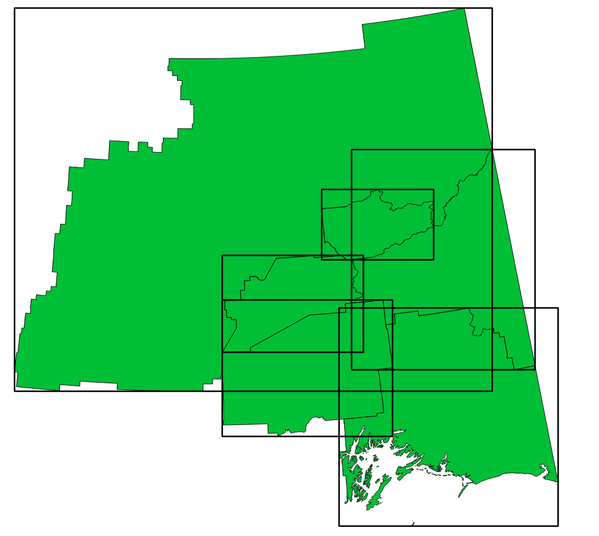Рис. 24.43 Black lines represent the bounding boxes of each polygon feature

См.также

Minimum bounding geometry

Parameters¶

Label

Имя

Тип

Описание

Input layer

INPUT

[vector: line, polygon]

Input line or polygon vector layer

Bounds

OUTPUT

[vector: polygon]

Default: [Create temporary layer]

Specify the output (bounding box) layer. One of:

• Create Temporary Layer (TEMPORARY_OUTPUT)

• Save to File…

• Save to Geopackage…

• Save to PostGIS Table…

The file encoding can also be changed here.

Outputs¶

Label

Имя

Тип

Описание

Bounds

OUTPUT

[vector: polygon]

Bounding boxes of input layer

Python code¶

Algorithm ID: native:boundingboxes

import processing
processing.run("algorithm_id", {parameter_dictionary})

The algorithm id is displayed when you hover over the algorithm in the Processing Toolbox. The parameter dictionary provides the parameter NAMEs and values. See Изпользование алгоритмов геообработки в консоли for details on how to run processing algorithms from the Python console.

24.1.16.6. Buffer¶

Computes a buffer area for all the features in an input layer, using a fixed distance.

It is possible to use a negative distance for polygon input layers. In this case the buffer will result in a smaller polygon (setback).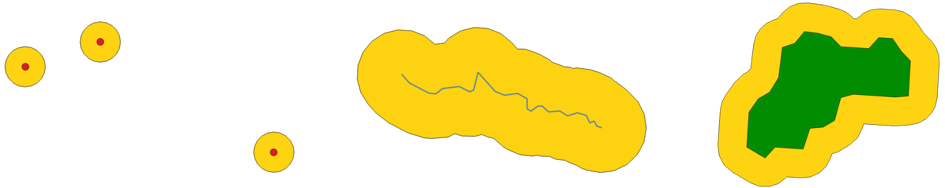Рис. 24.44 Buffer (in yellow) of points, line and polygon

Default menu: Vector ► Geoprocessing Tools

Parameters¶

Label

Имя

Тип

Описание

Input layer

INPUT

[vector: any]

Input vector layer

Distance

DISTANCE

Default: 10.0

Buffer distance (from the boundary of each feature). You can use the Data Defined button on the right to choose a field from which the radius will be calculated. This way you can have different radius for each feature (see Variable distance buffer).

Segments

SEGMENTS

[number]

Default: 5

Controls the number of line segments to use to approximate a quarter circle when creating rounded offsets.

End cap style

END_CAP_STYLE

[enumeration]

Default: 0

Controls how line endings are handled in the buffer. One of:

• 0 — Round

• 1 — Flat

• 2 — Square

Join style

JOIN_STYLE

[enumeration]

Default: 0

Specifies whether round, miter or beveled joins should be used when offsetting corners in a line. Options are:

• 0 — Round

• 1 — Miter

• 2 — Bevel

Miter limit

MITER_LIMIT

[number]

Default: 2.0

Controls the maximum distance from the offset curve to use when creating a mitered join (only applicable for miter join styles). Minimum: 1.

Dissolve result

DISSOLVE

[boolean]

Default: False

Dissolve the final buffer. If True (checked), overlapping buffers will be dissolved (combined) into a new feature.

Buffered

OUTPUT

[vector: polygon]

Default: [Create temporary layer]

Specify the output (buffer) layer. One of:

• Create Temporary Layer (TEMPORARY_OUTPUT)

• Save to File…

• Save to Geopackage…

• Save to PostGIS Table…

The file encoding can also be changed here.

Outputs¶

Label

Имя

Тип

Описание

Buffered

OUTPUT

[vector: polygon]

Output (buffer) polygon layer

Python code¶

Algorithm ID: native:buffer

import processing
processing.run("algorithm_id", {parameter_dictionary})

The algorithm id is displayed when you hover over the algorithm in the Processing Toolbox. The parameter dictionary provides the parameter NAMEs and values. See Изпользование алгоритмов геообработки в консоли for details on how to run processing algorithms from the Python console.

24.1.16.7. Centroids¶

Creates a new point layer, with points representing the centroids of the geometries of the input layer.

The centroid is a single point representing the barycenter (of all parts) of the feature, so it can be outside the feature borders. But can also be a point on each part of the feature.

The attributes of the points in the output layer are the same as for the original features.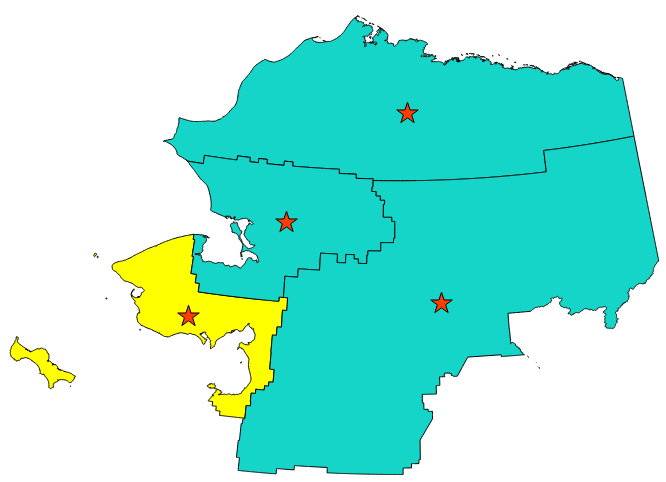Рис. 24.47 The red stars represent the centroids of the features of the input layer.

Default menu: Vector ► Geometry Tools

См.также

Point on Surface

Parameters¶

Label

Имя

Тип

Описание

Input layer

INPUT

[vector: any]

Input vector layer

Create centroid for each part

ALL_PARTS

Default: False

If True (checked), a centroid will be created for each part of the geometry

Centroids

OUTPUT

[vector: point]

Default: [Create temporary layer]

Specify the output (centroid) layer. One of:

• Create Temporary Layer (TEMPORARY_OUTPUT)

• Save to File…

• Save to Geopackage…

• Save to PostGIS Table…

The file encoding can also be changed here.

Outputs¶

Label

Имя

Тип

Описание

Centroids

OUTPUT

[vector: point]

Output point vector layer (centroids)

Python code¶

Algorithm ID: native:centroids

import processing
processing.run("algorithm_id", {parameter_dictionary})

The algorithm id is displayed when you hover over the algorithm in the Processing Toolbox. The parameter dictionary provides the parameter NAMEs and values. See Изпользование алгоритмов геообработки в консоли for details on how to run processing algorithms from the Python console.

24.1.16.8. Check validity¶

Performs a validity check on the geometries of a vector layer.

The geometries are classified in three groups (valid, invalid and error) and for each group, a vector layer with its features is generated:

• The Valid output layer contains only the valid features (without topological errors).

• The Invalid output layer contains all the invalid features found by the algorithm.

• The Error output layer is a point layer that points to where the invalid features were found.

The attribute tables of the generated layers will contain some additional information («message» for the error layer, «FID» and «_errors» for the invalid layer and only «FID» for the valid layer):

The attribute table of each generated vector layer will contain some additional information (number of errors found and types of error):Рис. 24.48 Left: the input layer. Right: the valid layer (green), the invalid layer (orange)

Default menu: Vector ► Geometry Tools

См.также

Fix geometries and the core plugin Geometry Checker Plugin

Parameters¶

Label

Имя

Тип

Описание

Input layer

INPUT_LAYER

[vector: any]

Input vector layer

Method

METHOD

[enumeration]

Default: 2

Method to use to check validity. Options:

• 0: The one selected in digitizing settings

• 1: QGIS

• 2: GEOS

Ignore ring self intersection

IGNORE_RING_SELF_INTERSECTION

[boolean]

Default: False

Ignore self intersecting rings when checking for validity.

Valid output

VALID_OUTPUT

[same as input]

Default: [Create temporary layer]

Specify the vector layer to contain a copy of the valid features of the source layer. One of:

• Skip output

• Create Temporary Layer (TEMPORARY_OUTPUT)

• Save to File…

• Save to Geopackage…

• Save to PostGIS Table…

The file encoding can also be changed here.

Invalid output

INVALID_OUTPUT

[same as input]

Default: [Create temporary layer]

Vector layer containing copy of the invalid features of the source layer with the field _errors listing the summary of the error(s) found. One of:

• Skip output

• Create Temporary Layer (TEMPORARY_OUTPUT)

• Save to File…

• Save to Geopackage…

• Save to PostGIS Table…

The file encoding can also be changed here.

Error output

ERROR_OUTPUT

[vector: point]

Default: [Create temporary layer]

Point layer of the exact position of the validity problems detected with the message field describing the error(s) found. One of:

• Skip output

• Create Temporary Layer (TEMPORARY_OUTPUT)

• Save to File…

• Save to Geopackage…

• Save to PostGIS Table…

The file encoding can also be changed here.

Outputs¶

Label

Имя

Тип

Описание

Count of errors

ERROR_COUNT

[number]

The number of geometries that caused errors.

Error output

ERROR_OUTPUT

[vector: point]

Point layer of the exact position of the validity problems detected with the message field describing the error(s) found.

Count of invalid features

INVALID_COUNT

[number]

The number of invalid geometries.

Invalid output

INVALID_OUTPUT

[same as input]

Vector layer containing copy of the invalid features of the source layer with the field _errors listing the summary of the error(s) found.

Count of valid features

VALID_COUNT

[number]

The number of valid geometries.

Valid output

VALID_OUTPUT

[same as input]

Vector layer containing a copy of the valid features of the source layer.

Python code¶

Algorithm ID: qgis:checkvalidity

import processing
processing.run("algorithm_id", {parameter_dictionary})

The algorithm id is displayed when you hover over the algorithm in the Processing Toolbox. The parameter dictionary provides the parameter NAMEs and values. See Изпользование алгоритмов геообработки в консоли for details on how to run processing algorithms from the Python console.

Types of error messages and their meanings¶

Таблица 24.1 If the GEOS method is used the following error messages can occur:

Error message

Explanation

Example

Repeated point

This error happens when a given vertex is repeated.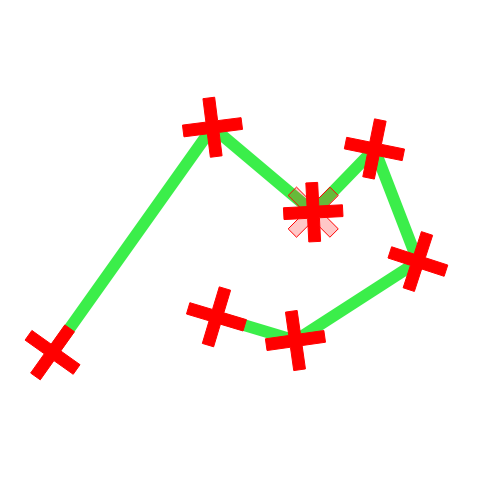Ring self-intersection

This error happens when a geometry touches itself and generates a ring.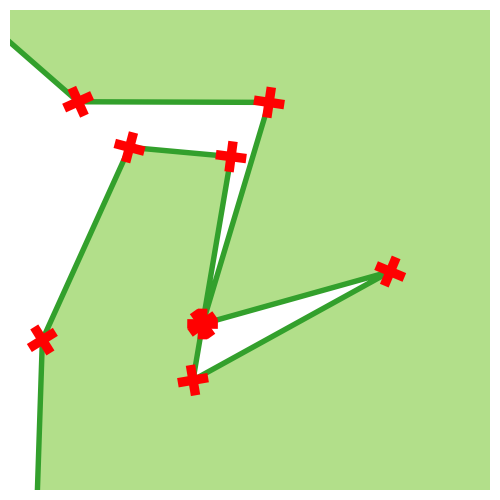Self-intersection

This error happens when a geometry touches itself.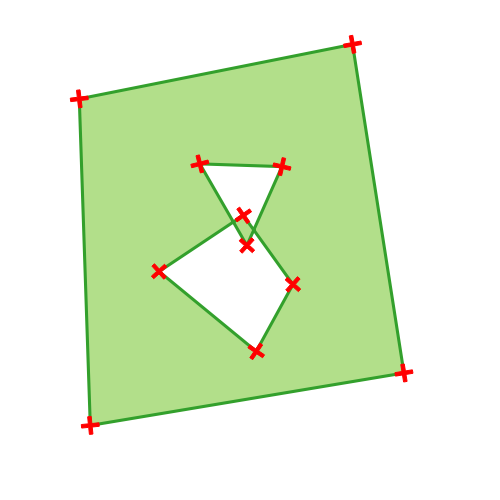Topology validation error

Hole lies outside shell

Holes are nested

Interior is disconnected

Nested shells

This error happens when a polygon geometry is on top of another polygon geometry.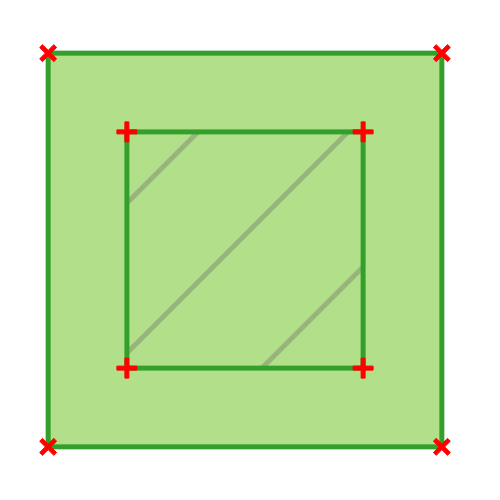Duplicate rings

This error happens when two rings (exterior or interior) of a polygon geometry are identical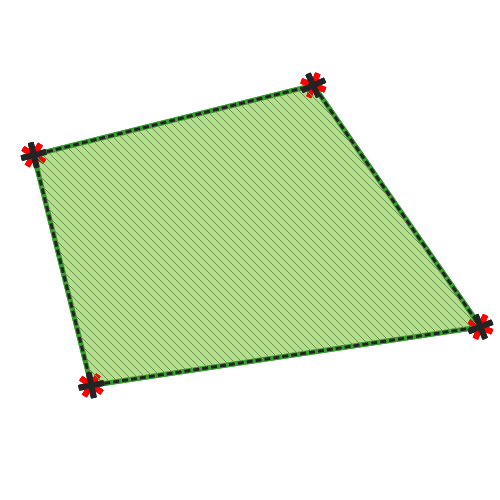Too few points in geometry component

Invalid coordinate

For a point geometry, this error happens when the geometry does not have a proper coordinate pair. The coordinate pair does not contain a latitude value and a longitude value in that order.

Ring is not closed

Таблица 24.2 If the QGIS method is used the following error messages can occur:

Error message

Explanation

Example

Segment %1 of ring %2 of polygon %3 intersects segment %4 of ring %5 of polygon %6 at %7

Ring %1 with less than four points

Ring %1 not closed

Line %1 with less than two points

Line %1 contains %n duplicate node(s) at %2

This error happens when consecutive points on a line have the same coordinates.Segments %1 and %2 of line %3 intersect at %4

This error happens when a line self intersects (two segments of the line intersect each other).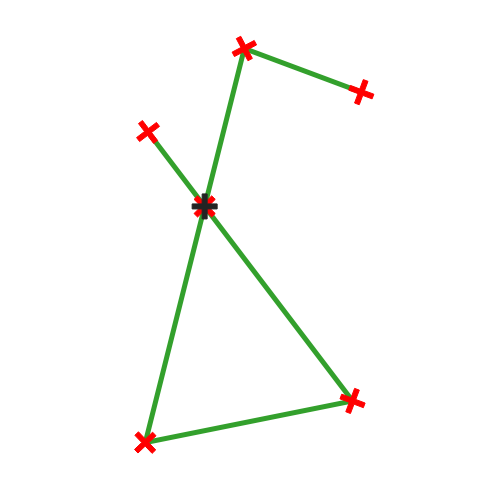Ring self-intersection

This error happens when an outer or inner (island) ring / boundary of a polygon geometry intersects itself.Ring %1 of polygon %2 not in exterior ring

Polygon %1 lies inside polygon %2

This error happens when a part of a MultiPolygon geometry is inside a hole of a MultiPolygon geometry.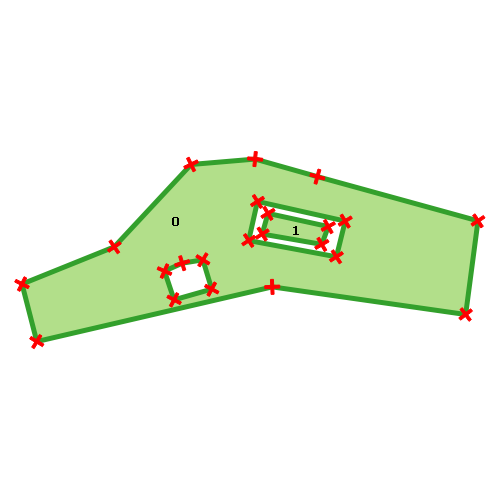24.1.16.9. Collect geometries¶

Takes a vector layer and collects its geometries into new multipart geometries.

One or more attributes can be specified to collect only geometries belonging to the same class (having the same value for the specified attributes), alternatively all geometries can be collected.

All output geometries will be converted to multi geometries, even those with just a single part. This algorithm does not dissolve overlapping geometries - they will be collected together without modifying the shape of each geometry part.

See the „Promote to multipart“ or „Aggregate“ algorithms for alternative options.

Default menu: Vector ► Geometry Tools

См.также

Parameters¶

Label

Имя

Тип

Описание

Input layer

INPUT

[vector: any]

Input vector layer

Unique ID fields

FIELD

[tablefield: any] [list]

Choose one or more attributes to collect the geometries

Collected

OUTPUT

[same as input]

Vector layer with collected geometries

Outputs¶

Label

Имя

Тип

Описание

Collected

OUTPUT

[same as input]

Default: [Create temporary layer]

Specify the output vector layer for the collected geometries. One of:

• Create Temporary Layer (TEMPORARY_OUTPUT)

• Save to File…

• Save to Geopackage…

• Save to PostGIS Table…

The file encoding can also be changed here.

Python code¶

Algorithm ID: native:collect

import processing
processing.run("algorithm_id", {parameter_dictionary})

The algorithm id is displayed when you hover over the algorithm in the Processing Toolbox. The parameter dictionary provides the parameter NAMEs and values. See Изпользование алгоритмов геообработки в консоли for details on how to run processing algorithms from the Python console.

24.1.16.10. Concave hull (alpha shapes)¶

Computes the concave hull of the features in an input point layer.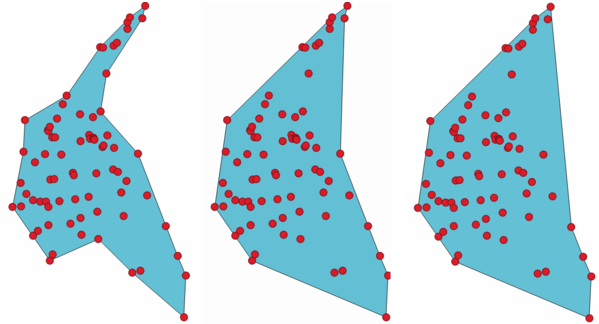Рис. 24.49 Concave hulls with different thresholds (0.3, 0.6, 0.9)

Parameters¶

Label

Имя

Тип

Описание

Input point layer

INPUT

[vector: point]

Input point vector layer

Threshold

ALPHA

[number]

Default: 0.3

Number from 0 (maximum concave hull) to 1 (convex hull).

Allow holes

HOLES

[boolean]

Default: True

Choose whether to allow holes in the final concave hull

Split multipart geometry into singlepart geometries

NO_MULTIGEOMETRY

[boolean]

Default: True

Check if you want to have singlepart geometries instead of multipart ones.

Concave hull

OUTPUT

[vector: polygon]

Default: [Create temporary layer]

Specify the output vector layer. One of:

• Create Temporary Layer (TEMPORARY_OUTPUT)

• Save to File…

• Save to Geopackage…

• Save to PostGIS Table…

The file encoding can also be changed here.

Outputs¶

Label

Имя

Тип

Описание

Concave hull

OUTPUT

[vector: polygon]

The output vector layer

Python code¶

Algorithm ID: qgis:concavehull

import processing
processing.run("algorithm_id", {parameter_dictionary})

The algorithm id is displayed when you hover over the algorithm in the Processing Toolbox. The parameter dictionary provides the parameter NAMEs and values. See Изпользование алгоритмов геообработки в консоли for details on how to run processing algorithms from the Python console.

24.1.16.11. Concave hull (k-nearest neighbor)¶

Generates a concave hull polygon from a set of points. If the input layer is a line or polygon layer, it will use the vertices.

The number of neighbors to consider determines the concaveness of the output polygon. A lower number will result in a concave hull that follows the points very closely, while a higher number will have a smoother shape. The minimum number of neighbor points to consider is 3. A value equal to or greater than the number of points will result in a convex hull.

If a field is selected, the algorithm will group the features in the input layer using unique values in that field and generate individual polygons in the output layer for each group.

См.также

Concave hull (alpha shapes)

Parameters¶

Label

Имя

Тип

Описание

Input layer

INPUT

[vector: any]

Input vector layer

Number of neighboring points to consider (a lower number is more concave, a higher number is smoother)

KNEIGHBORS

[number]

Default: 3

Determines the concaveness of the output polygon. A small number will result in a concave hull that follows the points very closely, while a high number will make the polygon look more like the convex hull (if the number is equal to or larger than the number of features, the result will be the convex hull). Minimum value: 3.

Field

Optional

FIELD

[tablefield: any]

Default: None

If specified, one concave hull polygon is generated for each unique value of the field (by selecting features using this value).

Concave hull

OUTPUT

[vector: polygon]

Default: [Create temporary layer]

Specify the output vector layer. One of:

• Create Temporary Layer (TEMPORARY_OUTPUT)

• Save to File…

• Save to Geopackage…

• Save to PostGIS Table…

The file encoding can also be changed here.

Outputs¶

Label

Имя

Тип

Описание

Concave hull

OUTPUT

[vector: polygon]

The output vector layer

Python code¶

Algorithm ID: qgis:knearestconcavehull

import processing
processing.run("algorithm_id", {parameter_dictionary})

The algorithm id is displayed when you hover over the algorithm in the Processing Toolbox. The parameter dictionary provides the parameter NAMEs and values. See Изпользование алгоритмов геообработки в консоли for details on how to run processing algorithms from the Python console.

24.1.16.12. Convert geometry type¶

Generates a new layer based on an existing one, with a different type of geometry.

The attribute table of the output layer is the same as the one of the input layer.

Not all conversions are possible. For instance, a line layer can be converted to a point layer, but a point layer cannot be converted to a line layer.

Parameters¶

Label

Имя

Тип

Описание

Input layer

INPUT

[vector: any]

Input vector layer

New geometry type

TYPE

[enumeration]

Default: 0

Geometry type to apply to the output features. One of:

• 0 — Centroids

• 1 — Nodes

• 2 — Linestrings

• 3 — Multilinestrings

• 4 — Polygons

Converted

OUTPUT

[vector: any]

Default: [Create temporary layer]

Specify the output vector layer. One of:

• Create Temporary Layer (TEMPORARY_OUTPUT)

• Save to File…

• Save to Geopackage…

• Save to PostGIS Table…

The file encoding can also be changed here.

Outputs¶

Label

Имя

Тип

Описание

Converted

OUTPUT

[vector: any]

Output vector layer - the type depends on the parameters

Python code¶

Algorithm ID: qgis:convertgeometrytype

import processing
processing.run("algorithm_id", {parameter_dictionary})

The algorithm id is displayed when you hover over the algorithm in the Processing Toolbox. The parameter dictionary provides the parameter NAMEs and values. See Изпользование алгоритмов геообработки в консоли for details on how to run processing algorithms from the Python console.

24.1.16.13. Convert to curved geometries¶

Converts a geometry into its curved geometry equivalent.

Already curved geometries will be retained without change.

Parameters¶

Label

Имя

Тип

Описание

Input layer

INPUT

[vector: line or polygon]

Input vector layer

Maximum distance tolerance

DISTANCE

[number]

Default: 0.000001

The maximum distance allowed between the original location of vertices and where they would fall on the converted curved geometries

Maximum angle tolerance

ANGLE

[number]

Default: 0.000001

Segments are considered as suitable for replacing with an arc if the points are all regularly spaced on the candidate arc. This parameter specifies the maximum angular deviation (in degrees) allowed when testing for regular point spacing. Between 0 and 45°.

Curves

OUTPUT

[vector: compoundcurve or curvepolygon]

Default: [Create temporary layer]

Specify the output vector layer. One of:

• Create Temporary Layer (TEMPORARY_OUTPUT)

• Save to File…

• Save to Geopackage…

• Save to Database Table…

• Append to Layer…

The file encoding can also be changed here.

Outputs¶

Label

Имя

Тип

Описание

Curves

OUTPUT

[vector: compoundcurve or curvepolygon]

Output vector layer with curved geometries

Python code¶

Algorithm ID: native:converttocurves

import processing
processing.run("algorithm_id", {parameter_dictionary})

The algorithm id is displayed when you hover over the algorithm in the Processing Toolbox. The parameter dictionary provides the parameter NAMEs and values. See Изпользование алгоритмов геообработки в консоли for details on how to run processing algorithms from the Python console.

24.1.16.14. Convex hull¶

Calculates the convex hull for each feature in an input layer.

See the „Minimum bounding geometry“ algorithm for a convex hull calculation which covers the whole layer or grouped subsets of features.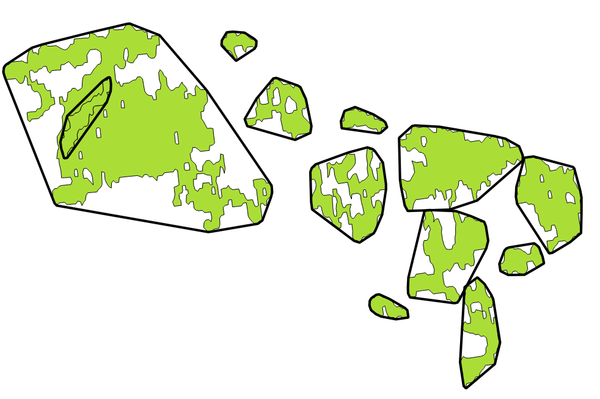Рис. 24.50 Black lines identify the convex hull for each layer feature

Default menu: Vector ► Geoprocessing Tools

Parameters¶

Label

Имя

Тип

Описание

Input layer

INPUT

[vector: any]

Input vector layer

Convex hull

OUTPUT

[vector: polygon]

Default: [Create temporary layer]

Specify the output vector layer. One of:

• Create Temporary Layer (TEMPORARY_OUTPUT)

• Save to File…

• Save to Geopackage…

• Save to PostGIS Table…

The file encoding can also be changed here.

Outputs¶

Label

Имя

Тип

Описание

Convex hull

OUTPUT

[vector: polygon]

The output (convex hull) vector layer

Python code¶

Algorithm ID: native:convexhull

import processing
processing.run("algorithm_id", {parameter_dictionary})

The algorithm id is displayed when you hover over the algorithm in the Processing Toolbox. The parameter dictionary provides the parameter NAMEs and values. See Изпользование алгоритмов геообработки в консоли for details on how to run processing algorithms from the Python console.

24.1.16.15. Create layer from extent¶

Creates a new vector layer that contains a single feature with geometry matching the extent of the input layer.

It can be used in models to convert a literal extent (xmin, xmax, ymin, ymax format) into a layer which can be used for other algorithms which require a layer based input.

См.также

Create layer from point

Parameters¶

Label

Имя

Тип

Описание

Extent (xmin, xmax, ymin, ymax)

INPUT

[extent]

Input extent

Extent

OUTPUT

[vector: polygon]

Default: [Create temporary layer]

Specify the output vector layer. One of:

• Create Temporary Layer (TEMPORARY_OUTPUT)

• Save to File…

• Save to Geopackage…

• Save to PostGIS Table…

The file encoding can also be changed here.

Outputs¶

Label

Имя

Тип

Описание

Extent

OUTPUT

[vector: polygon]

The output (extent) vector layer

Python code¶

Algorithm ID: native:extenttolayer

import processing
processing.run("algorithm_id", {parameter_dictionary})

The algorithm id is displayed when you hover over the algorithm in the Processing Toolbox. The parameter dictionary provides the parameter NAMEs and values. See Изпользование алгоритмов геообработки в консоли for details on how to run processing algorithms from the Python console.

24.1.16.16. Create layer from point¶

Creates a new vector layer that contains a single feature with geometry matching a point parameter. It can be used in models to convert a point into a point layer for algorithms which require a layer based input.

См.также

Create layer from extent

Parameters¶

Label

Имя

Тип

Описание

Point

INPUT

[coordinates]

Input point, including CRS info (example: 397254,6214446 [EPSG:32632]).

If the CRS is not provided, the Project CRS will be used.

The point can be specified by clicking on the map canvas.

Point

OUTPUT

[vector: point]

Default: [Create temporary layer]

Specify the output layer. One of:

• Create Temporary Layer (TEMPORARY_OUTPUT)

• Save to File…

• Save to Geopackage…

• Save to PostGIS Table…

The file encoding can also be changed here.

Outputs¶

Label

Имя

Тип

Описание

Point

OUTPUT

[vector: point]

The output point vector layer containing the input point.

Python code¶

Algorithm ID: native:pointtolayer

import processing
processing.run("algorithm_id", {parameter_dictionary})

The algorithm id is displayed when you hover over the algorithm in the Processing Toolbox. The parameter dictionary provides the parameter NAMEs and values. See Изпользование алгоритмов геообработки в консоли for details on how to run processing algorithms from the Python console.

24.1.16.17. Create wedge buffers¶

Creates wedge shaped buffers from input points.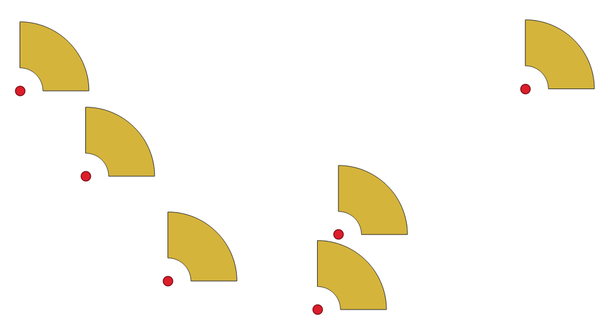Рис. 24.51 Wedge buffers

The native output from this algorithm are CurvePolygon geometries, but these may be automatically segmentized to Polygons depending on the output format.

Parameters¶

Label

Имя

Тип

Описание

Input layer

INPUT

[vector: point]

Input point vector layer

Azimuth (degrees from North)

AZIMUTH

Default: 0.0

Angle (in degrees) as the middle value of the wedge

Wedge width (in degrees)

WIDTH

Default: 45.0

Width (in degrees) of the buffer. The wedge will extend to half of the angular width either side of the azimuth direction.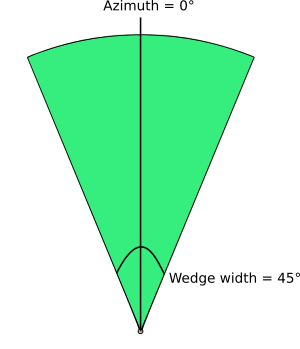Рис. 24.52 Azimuth and width values of the wedge buffer

Default: 1.0

The outer size (length) of the wedge: the size is meant from the source point to the edge of the wedge shape.

Optional

Default: 0.0

Inner radius value. If 0 the wedge will begin from the source point.

Buffers

OUTPUT

[vector: polygon]

Default: [Create temporary layer]

Specify the output vector layer. One of:

• Create Temporary Layer (TEMPORARY_OUTPUT)

• Save to File…

• Save to Geopackage…

• Save to PostGIS Table…

The file encoding can also be changed here.

Outputs¶

Label

Имя

Тип

Описание

Buffers

OUTPUT

[vector: polygon]

The output (wedge buffer) vector layer

Python code¶

Algorithm ID: native:wedgebuffers

import processing
processing.run("algorithm_id", {parameter_dictionary})

The algorithm id is displayed when you hover over the algorithm in the Processing Toolbox. The parameter dictionary provides the parameter NAMEs and values. See Изпользование алгоритмов геообработки в консоли for details on how to run processing algorithms from the Python console.

24.1.16.18. Delaunay triangulation¶

Creates a polygon layer with the Delaunay triangulation corresponding to the input point layer.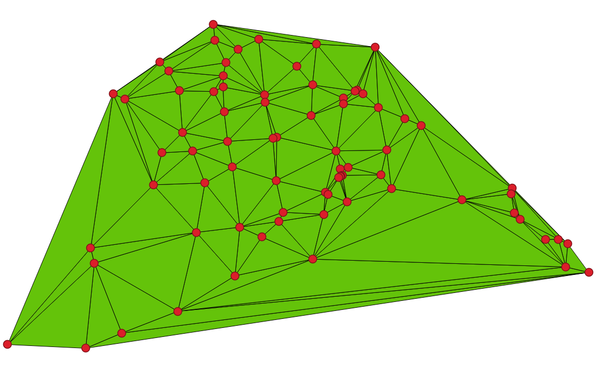Рис. 24.53 Delaunay triangulation on points

Default menu: Vector ► Geometry Tools

Parameters¶

Label

Имя

Тип

Описание

Input layer

INPUT

[vector: point]

Input point vector layer

Delaunay triangulation

OUTPUT

[vector: polygon]

Default: [Create temporary layer]

Specify the output vector layer. One of:

• Create Temporary Layer (TEMPORARY_OUTPUT)

• Save to File…

• Save to Geopackage…

• Save to PostGIS Table…

The file encoding can also be changed here.

Outputs¶

Label

Имя

Тип

Описание

Delaunay triangulation

OUTPUT

[vector: polygon]

The output (Delaunay triangulation) vector layer

Python code¶

Algorithm ID: qgis:delaunaytriangulation

import processing
processing.run("algorithm_id", {parameter_dictionary})

The algorithm id is displayed when you hover over the algorithm in the Processing Toolbox. The parameter dictionary provides the parameter NAMEs and values. See Изпользование алгоритмов геообработки в консоли for details on how to run processing algorithms from the Python console.

24.1.16.19. Delete holes¶

Takes a polygon layer and removes holes in polygons. It creates a new vector layer in which polygons with holes have been replaced by polygons with only their external ring. Attributes are not modified.

An optional minimum area parameter allows removing only holes which are smaller than a specified area threshold. Leaving this parameter at 0.0 results in all holes being removed.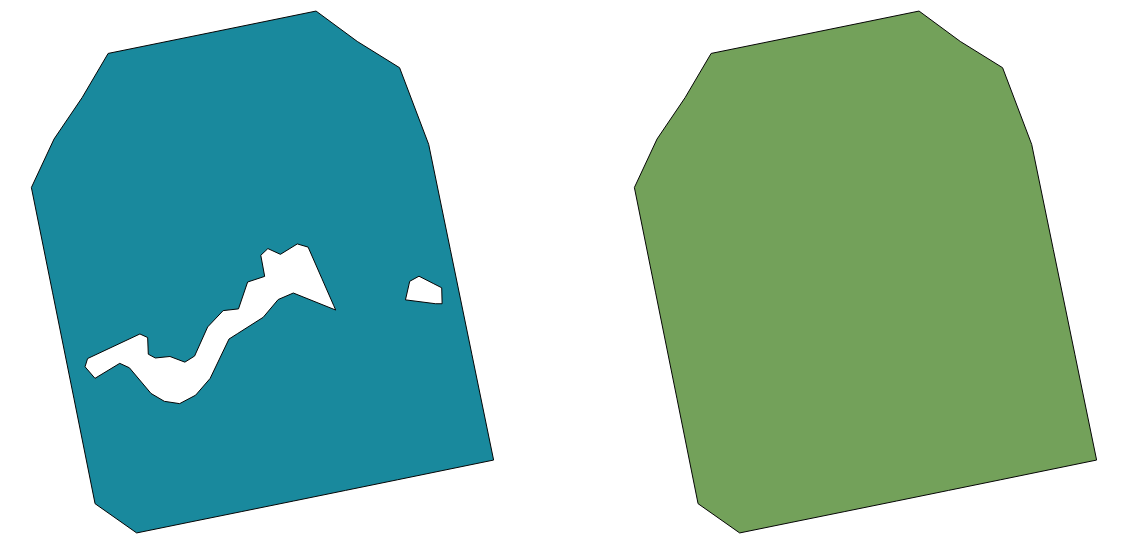Рис. 24.54 Before and after the cleaning

Parameters¶

Label

Имя

Тип

Описание

Input layer

INPUT

[vector: polygon]

Input polygon vector layer

Remove holes with area less than

Optional

MIN_AREA

Default: 0.0

Only holes with an area less than this threshold will be deleted. With a value of 0.0, all the holes will be deleted.

Cleaned

OUTPUT

[same as input]

Default: [Create temporary layer]

Specify the output vector layer. One of:

• Create Temporary Layer (TEMPORARY_OUTPUT)

• Save to File…

• Save to Geopackage…

• Save to PostGIS Table…

The file encoding can also be changed here.

Outputs¶

Label

Имя

Тип

Описание

Cleaned

OUTPUT

[same as input]

The output (cleaned) vector layer

Python code¶

Algorithm ID: native:deleteholes

import processing
processing.run("algorithm_id", {parameter_dictionary})

The algorithm id is displayed when you hover over the algorithm in the Processing Toolbox. The parameter dictionary provides the parameter NAMEs and values. See Изпользование алгоритмов геообработки в консоли for details on how to run processing algorithms from the Python console.

24.1.16.20. Densify by count¶

Takes a polygon or line layer and generates a new one in which the geometries have a larger number of vertices than the original one.

If the geometries have Z or M values present then these will be linearly interpolated at the added vertices.

The number of new vertices to add to each segment is specified as an input parameter.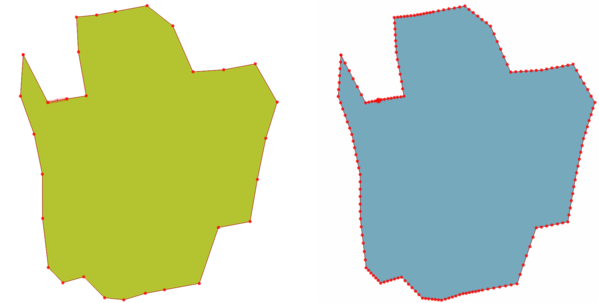Рис. 24.55 Red points show the vertices before and after the densify

Default menu: Vector ► Geometry Tools

См.также

Densify by interval

Parameters¶

Label

Имя

Тип

Описание

Input layer

INPUT

[vector: line, polygon]

Input line or polygon vector layer

VERTICES

[number]

Default: 1

Number of vertices to add to each segment

Densified

OUTPUT

[same as input]

Default: [Create temporary layer]

Specify the output vector layer. One of:

• Create Temporary Layer (TEMPORARY_OUTPUT)

• Save to File…

• Save to Geopackage…

• Save to PostGIS Table…

The file encoding can also be changed here.

Outputs¶

Label

Имя

Тип

Описание

Densified

OUTPUT

[same as input]

The output (densified) vector layer

Python code¶

Algorithm ID: native:densifygeometries

import processing
processing.run("algorithm_id", {parameter_dictionary})

The algorithm id is displayed when you hover over the algorithm in the Processing Toolbox. The parameter dictionary provides the parameter NAMEs and values. See Изпользование алгоритмов геообработки в консоли for details on how to run processing algorithms from the Python console.

24.1.16.21. Densify by interval¶

Takes a polygon or line layer and generates a new one in which the geometries have a larger number of vertices than the original one.

The geometries are densified by adding regularly placed extra vertices inside each segment so that the maximum distance between any two vertices does not exceed the specified distance.

If the geometries have Z or M values present then these will be linearly interpolated at the added vertices.

Example

Specifying a distance of 3 would cause the segment [0 0] -> [10 0] to be converted to [0 0] -> [2.5 0] -> [5 0] -> [7.5 0] -> [10 0], since 3 extra vertices are required on the segment and spacing these at 2.5 increments allows them to be evenly spaced over the segment.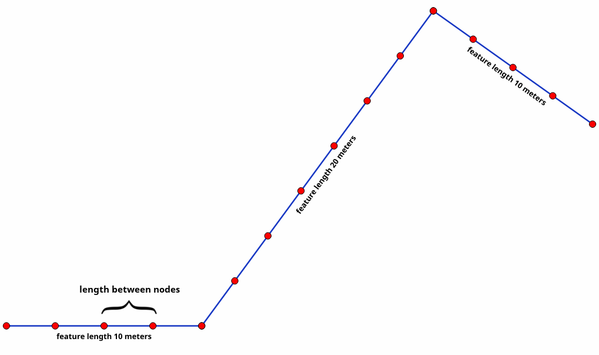Рис. 24.56 Densify geometry at a given interval

См.также

Densify by count

Parameters¶

Label

Имя

Тип

Описание

Input layer

INPUT

[vector: line, polygon]

Input line or polygon vector layer

INTERVAL

Default: 1.0

Maximum distance between two consecutive vertices

Densified

OUTPUT

[same as input]

Default: [Create temporary layer]

Specify the output vector layer. One of:

• Create Temporary Layer (TEMPORARY_OUTPUT)

• Save to File…

• Save to Geopackage…

• Save to PostGIS Table…

The file encoding can also be changed here.

Outputs¶

Label

Имя

Тип

Описание

Densified

OUTPUT

[same as input]

The output (densified) vector layer

Python code¶

Algorithm ID: native:densifygeometriesgivenaninterval

import processing
processing.run("algorithm_id", {parameter_dictionary})

The algorithm id is displayed when you hover over the algorithm in the Processing Toolbox. The parameter dictionary provides the parameter NAMEs and values. See Изпользование алгоритмов геообработки в консоли for details on how to run processing algorithms from the Python console.

24.1.16.22. Dissolve¶

Takes a vector layer and combines its features into new features. One or more attributes can be specified to dissolve features belonging to the same class (having the same value for the specified attributes), alternatively all features can be dissolved to a single feature.

All output geometries will be converted to multi geometries. In case the input is a polygon layer, common boundaries of adjacent polygons being dissolved will get erased.

The resulting attribute table will have the same fields as the input layer. The values in the output layer’s fields are the ones of the first input feature that happens to be processed.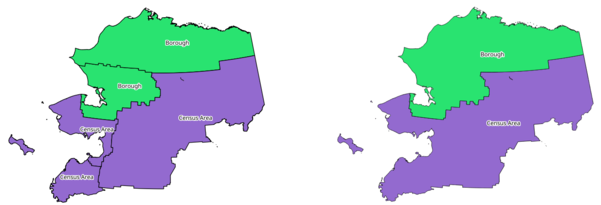Рис. 24.57 Dissolve the polygon layer on a common attribute

Default menu: Vector ► Geoprocessing Tools

См.также

Parameters¶

Label

Имя

Тип

Описание

Input layer

INPUT

[vector: any]

Input vector layer

Dissolve field(s)

Optional

FIELD

[tablefield: any] [list]

Default: []

Features having the same value for the selected field(s) will be replaced with a single one and their geometries are merged.

If no field is provided then all the features are dissolved, resulting in a single (multipart) feature.

Dissolved

OUTPUT

[same as input]

Default: [Create temporary layer]

Specify the output vector layer. One of:

• Create Temporary Layer (TEMPORARY_OUTPUT)

• Save to File…

• Save to Geopackage…

• Save to PostGIS Table…

The file encoding can also be changed here.

Outputs¶

Label

Имя

Тип

Описание

Dissolved

OUTPUT

[same as input]

The output vector layer with dissolved geometries

Python code¶

Algorithm ID: native:dissolve

import processing
processing.run("algorithm_id", {parameter_dictionary})

The algorithm id is displayed when you hover over the algorithm in the Processing Toolbox. The parameter dictionary provides the parameter NAMEs and values. See Изпользование алгоритмов геообработки в консоли for details on how to run processing algorithms from the Python console.

24.1.16.23. Drape (set Z value from raster)¶

Uses values sampled from a band within a raster layer to set the Z value for every overlapping vertex in the feature geometry. The raster values can optionally be scaled by a preset amount.

If Z values already exist in the layer, they will be overwritten with the new value. If no Z values exist, the geometry will be upgraded to include the Z dimension.

См.также

Parameters¶

Label

Имя

Тип

Описание

Input layer

INPUT

[vector: any]

Input vector layer

Raster layer

RASTER

[raster]

Raster layer with Z values

Band number

BAND

[raster band]

Default: 1

The raster band to take the Z values from

Value for nodata or non-intersecting vertices

NODATA

Default: 0

Value to use in case the vertex does not intersect (a valid pixel of) the raster

Scale factor

SCALE

Default: 1.0

Scaling value: the band values are multiplied by this value.

Updated

OUTPUT

[same as input]

Default: [Create temporary layer]

Specify the output vector layer (with Z values from the raster layer). One of:

• Create Temporary Layer (TEMPORARY_OUTPUT)

• Save to File…

• Save to Geopackage…

• Save to PostGIS Table…

The file encoding can also be changed here.

Outputs¶

Label

Имя

Тип

Описание

Updated

OUTPUT

[same as input]

The output vector layer with Z values from the raster layer

Python code¶

Algorithm ID: native:setzfromraster

import processing
processing.run("algorithm_id", {parameter_dictionary})

The algorithm id is displayed when you hover over the algorithm in the Processing Toolbox. The parameter dictionary provides the parameter NAMEs and values. See Изпользование алгоритмов геообработки в консоли for details on how to run processing algorithms from the Python console.

24.1.16.24. Drop M/Z values¶

Removes M (measure) or Z (altitude) values from input geometries.

См.также

Parameters¶

Label

Имя

Тип

Описание

Input layer

INPUT

[vector: any]

Input vector layer with M or Z values

Drop M Values

DROP_M_VALUES

[boolean]

Default: False

Removes the M values from the geometries

Drop Z Values

DROP_Z_VALUES

[boolean]

Default: False

Removes the Z values from the geometries

Z/M Dropped

OUTPUT

[same as input]

Default: [Create temporary layer]

Specify the output vector layer. One of:

• Create Temporary Layer (TEMPORARY_OUTPUT)

• Save to File…

• Save to Geopackage…

• Save to PostGIS Table…

The file encoding can also be changed here.

Outputs¶

Label

Имя

Тип

Описание

Z/M Dropped

OUTPUT

[same as input]

The output vector layer (identical to the input layer, except that the M and/or Z dimensions have been removed from the geometries).

Python code¶

Algorithm ID: native:dropmzvalues

import processing
processing.run("algorithm_id", {parameter_dictionary})

The algorithm id is displayed when you hover over the algorithm in the Processing Toolbox. The parameter dictionary provides the parameter NAMEs and values. See Изпользование алгоритмов геообработки в консоли for details on how to run processing algorithms from the Python console.

24.1.16.25. Eliminate selected polygons¶

Combines selected polygons of the input layer with certain adjacent polygons by erasing their common boundary. The adjacent polygon can be either the one with the largest or smallest area or the one sharing the largest common boundary with the polygon to be eliminated.

Eliminate is normally used to get rid of sliver polygons, i.e. tiny polygons that are a result of polygon intersection processes where boundaries of the inputs are similar but not identical.

Default menu: Vector ► Geoprocessing Tools

См.также

Fix geometries

Parameters¶

Label

Имя

Тип

Описание

Input layer

INPUT

[vector: polygon]

Input polygon vector layer

Merge selection with the neighboring polygon with the

MODE

[enumeration]

Default: None

Choose the parameter to use in order to get rid of the selected polygons:

• 0 — Largest Area

• 1 — Smallest Area

• 2 — Largest Common Boundary

Eliminated

OUTPUT

[vector: polygon]

Default: [Create temporary layer]

Specify the output vector layer. One of:

• Create Temporary Layer (TEMPORARY_OUTPUT)

• Save to File…

• Save to Geopackage…

• Save to PostGIS Table…

The file encoding can also be changed here.

Outputs¶

Label

Имя

Тип

Описание

Eliminated

OUTPUT

[vector: polygon]

The output polygon vector layer.

Python code¶

Algorithm ID: qgis:eliminateselectedpolygons

import processing
processing.run("algorithm_id", {parameter_dictionary})

The algorithm id is displayed when you hover over the algorithm in the Processing Toolbox. The parameter dictionary provides the parameter NAMEs and values. See Изпользование алгоритмов геообработки в консоли for details on how to run processing algorithms from the Python console.

24.1.16.26. Explode lines¶

Takes a lines layer and creates a new one in which each line layer is replaced by a set of lines representing the segments in the original line.

Each line in the resulting layer contains only a start and an end point, with no intermediate vertices between them.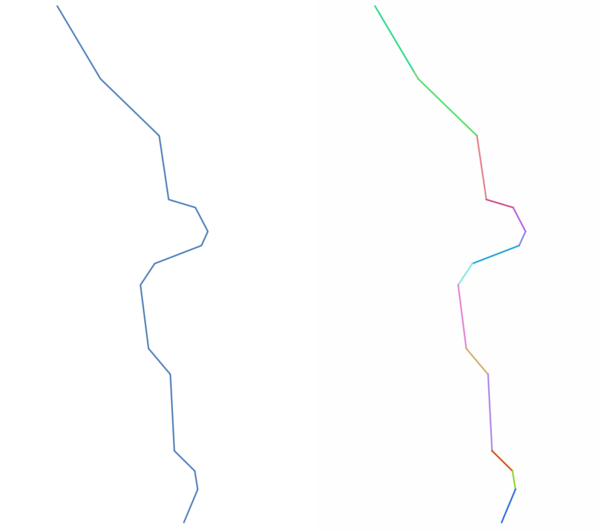Рис. 24.58 The original line layer and the exploded one

См.также

Parameters¶

Label

Имя

Тип

Описание

Input layer

INPUT

[vector: line]

Input line vector layer

Exploded

OUTPUT

[vector: line]

Default: [Create temporary layer]

Specify the output vector layer. One of:

• Create Temporary Layer (TEMPORARY_OUTPUT)

• Save to File…

• Save to Geopackage…

• Save to PostGIS Table…

The file encoding can also be changed here.

Outputs¶

Label

Имя

Тип

Описание

Exploded

OUTPUT

[vector: line]

The output line vector layer with features representing each segment of the input layer.

Python code¶

Algorithm ID: native:explodelines

import processing
processing.run("algorithm_id", {parameter_dictionary})

The algorithm id is displayed when you hover over the algorithm in the Processing Toolbox. The parameter dictionary provides the parameter NAMEs and values. See Изпользование алгоритмов геообработки в консоли for details on how to run processing algorithms from the Python console.

24.1.16.27. Extend lines¶

Extends line geometry by a specified amount at the start and end of the line.

Lines are extended using the bearing of the first and last segment in the line.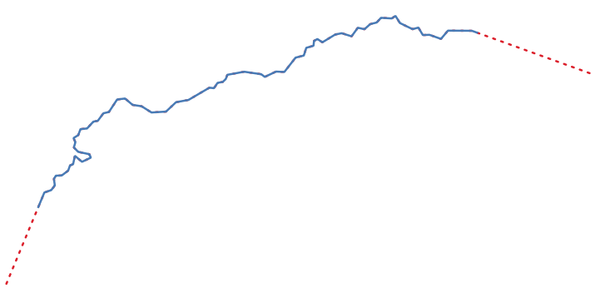Рис. 24.59 The red dashes represent the initial and final extension of the original layer

См.также

Line substring

Parameters¶

Label

Имя

Тип

Описание

Input layer

INPUT

[vector: line]

Input line vector layer

Start distance

START_DISTANCE

Distance by which to extend the first segment of the line (starting point)

End distance

END_DISTANCE

Distance by which to extend the last segment of the line (ending point)

Extended

OUTPUT

[vector: line]

Default: [Create temporary layer]

Specify the output vector layer. One of:

• Create Temporary Layer (TEMPORARY_OUTPUT)

• Save to File…

• Save to Geopackage…

• Save to PostGIS Table…

The file encoding can also be changed here.

Outputs¶

Label

Имя

Тип

Описание

Extended

OUTPUT

[vector: line]

The output (extended) line vector layer.

Python code¶

Algorithm ID: native:extendlines

import processing
processing.run("algorithm_id", {parameter_dictionary})

The algorithm id is displayed when you hover over the algorithm in the Processing Toolbox. The parameter dictionary provides the parameter NAMEs and values. See Изпользование алгоритмов геообработки в консоли for details on how to run processing algorithms from the Python console.

24.1.16.28. Extract M values¶

Extracts M values from geometries into feature attributes.

By default only the M value from the first vertex of each feature is extracted, however the algorithm can optionally calculate statistics on all of the geometry’s M values, including sum, mean, minimum and maximum.

См.также

Parameters¶

Label

Имя

Тип

Описание

Input layer

INPUT

[vector: any]

Input vector layer

Summaries to calculate

SUMMARIES

[enumeration]

Default: 

Statistics on the M values of a geometry. One or more of:

• 0 — First

• 1 — Last

• 2 — Count

• 3 — Sum

• 4 — Mean

• 5 — Median

• 6 — St.dev (pop)

• 7 — Minimum

• 8 — Maximum

• 9 — Range

• 10 — Minority

• 11 — Majority

• 12 — Variety

• 13 — Q1

• 14 — Q3

• 15 — IQR

Output column prefix

COLUMN_PREFIX

[string]

Default: „m_“

The prefix for the output (M) column

Extracted

OUTPUT

[same as input]

Default: [Create temporary layer]

Specify the output layer. One of:

• Create Temporary Layer (TEMPORARY_OUTPUT)

• Save to File…

• Save to Geopackage…

• Save to PostGIS Table…

The file encoding can also be changed here.

Outputs¶

Label

Имя

Тип

Описание

Extracted

OUTPUT

[same as input]

The output vector layer (with M values)

Python code¶

Algorithm ID: native:extractmvalues

import processing
processing.run("algorithm_id", {parameter_dictionary})

The algorithm id is displayed when you hover over the algorithm in the Processing Toolbox. The parameter dictionary provides the parameter NAMEs and values. See Изпользование алгоритмов геообработки в консоли for details on how to run processing algorithms from the Python console.

24.1.16.29. Extract specific vertices¶

Takes a vector layer and generates a point layer with points representing specific vertices in the input geometries.

For instance, this algorithm can be used to extract the first or last vertices in the geometry. The attributes associated to each point are the same ones associated to the feature that the vertex belongs to.

The vertex indices parameter accepts a comma separated string specifying the indices of the vertices to extract. The first vertex corresponds to an index of 0, the second vertex has an index of 1, etc. Negative indices can be used to find vertices at the end of the geometry, e.g., an index of -1 corresponds to the last vertex, -2 corresponds to the second last vertex, etc.

Additional fields are added to the vertices indicating the specific vertex position (e.g., 0, -1, etc), the original vertex index, the vertex’s part and its index within the part (as well as its ring for polygons), distance along the original geometry and bisector angle of vertex for the original geometry.

Parameters¶

Label

Имя

Тип

Описание

Input layer

INPUT

[vector: any]

Input vector layer

Vertex indices

VERTICES

[string]

Default: „0“

Comma-separated string of the indices of the vertices to extract.

Vertices

OUTPUT

[vector: point]

Default: [Create temporary layer]

Specify the output vector layer. One of:

• Create Temporary Layer (TEMPORARY_OUTPUT)

• Save to File…

• Save to Geopackage…

• Save to PostGIS Table…

The file encoding can also be changed here.

Outputs¶

Label

Имя

Тип

Описание

Vertices

OUTPUT

[vector: point]

The output (point) vector layer containing the specified vertices from the input layer geometries.

Python code¶

Algorithm ID: native:extractspecificvertices

import processing
processing.run("algorithm_id", {parameter_dictionary})

The algorithm id is displayed when you hover over the algorithm in the Processing Toolbox. The parameter dictionary provides the parameter NAMEs and values. See Изпользование алгоритмов геообработки в консоли for details on how to run processing algorithms from the Python console.

24.1.16.30. Extract vertices¶

Takes a vector layer and generates a point layer with points representing the vertices in the input geometries.

The attributes associated to each point are the same ones associated to the feature that the vertex belongs to.

Additional fields are added to the vertices indicating the vertex index (beginning at 0), the feature’s part and its index within the part (as well as its ring for polygons), distance along original geometry and bisector angle of vertex for original geometry.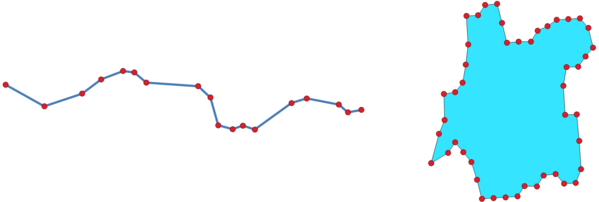Рис. 24.60 Vertices extracted for line and polygon layer

Default menu: Vector ► Geometry Tools

Parameters¶

Label

Имя

Тип

Описание

Input layer

INPUT

[vector: any]

Input vector layer

Vertices

OUTPUT

[vector: point]

Default: [Create temporary layer]

Specify the output vector layer. One of:

• Create Temporary Layer (TEMPORARY_OUTPUT)

• Save to File…

• Save to Geopackage…

• Save to PostGIS Table…

The file encoding can also be changed here.

Outputs¶

Label

Имя

Тип

Описание

Vertices

OUTPUT

[vector: point]

The output (point) vector layer containing the vertices from the input layer geometries.

Python code¶

Algorithm ID: native:extractvertices

import processing
processing.run("algorithm_id", {parameter_dictionary})

The algorithm id is displayed when you hover over the algorithm in the Processing Toolbox. The parameter dictionary provides the parameter NAMEs and values. See Изпользование алгоритмов геообработки в консоли for details on how to run processing algorithms from the Python console.

24.1.16.31. Extract Z values¶

Extracts Z values from geometries into feature attributes.

By default only the Z value from the first vertex of each feature is extracted, however the algorithm can optionally calculate statistics on all of the geometry’s Z values, including sum, mean, minimum and maximum.

См.также

Parameters¶

Label

Имя

Тип

Описание

Input layer

INPUT

[vector: any]

Input vector layer

Summaries to calculate

SUMMARIES

[enumeration]

Default: 

Statistics on the Z values of a geometry. One or more of:

• 0 — First

• 1 — Last

• 2 — Count

• 3 — Sum

• 4 — Mean

• 5 — Median

• 6 — St.dev (pop)

• 7 — Minimum

• 8 — Maximum

• 9 — Range

• 10 — Minority

• 11 — Majority

• 12 — Variety

• 13 — Q1

• 14 — Q3

• 15 — IQR

Output column prefix

COLUMN_PREFIX

[string]

Default: „z_“

The prefix for the output (Z) column

Extracted

OUTPUT

[same as input]

Default: [Create temporary layer]

Specify the output layer. One of:

• Create Temporary Layer (TEMPORARY_OUTPUT)

• Save to File…

• Save to Geopackage…

• Save to PostGIS Table…

The file encoding can also be changed here.

Outputs¶

Label

Имя

Тип

Описание

Extracted

OUTPUT

[same as input]

The output vector layer (with Z values)

Python code¶

Algorithm ID: native:extractzvalues

import processing
processing.run("algorithm_id", {parameter_dictionary})

The algorithm id is displayed when you hover over the algorithm in the Processing Toolbox. The parameter dictionary provides the parameter NAMEs and values. See Изпользование алгоритмов геообработки в консоли for details on how to run processing algorithms from the Python console.

24.1.16.32. Filter vertices by M value¶

Filters away vertices based on their M value, returning geometries with only vertex points that have a M value greater than or equal to the specified minimum value and/or less than or equal to the maximum value.

If the minimum value is not specified then only the maximum value is tested, and similarly if the maximum value is not specified then only the minimum value is tested.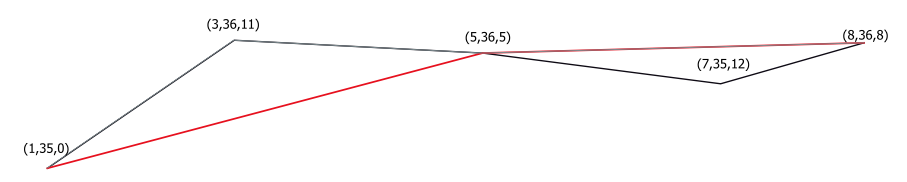Рис. 24.61 The red line represents the black line with only vertices whose M value is <=10.

Примечание

Depending on the input geometry attributes and the filters used, the resultant geometries created by this algorithm may no longer be valid.

Parameters¶

Label

Имя

Тип

Описание

Input layer

INPUT

[vector: line, polygon]

Input line or polygon vector layer to remove vertices from

Minimum

Optional

MIN

Default: Not set

Minimum of M values allowed

Maximum

Optional

MAX

Default: Not set

Maximum of M values allowed

Filtered

OUTPUT

[same as input]

Default: [Create temporary layer]

Specify the output vector layer. One of:

• Create Temporary Layer (TEMPORARY_OUTPUT)

• Save to File…

• Save to Geopackage…

• Save to PostGIS Table…

The file encoding can also be changed here.

Outputs¶

Label

Имя

Тип

Описание

Filtered

OUTPUT

[same as input]

The output vector layer of features with only the filtered vertices.

Python code¶

Algorithm ID: native:filterverticesbym

import processing
processing.run("algorithm_id", {parameter_dictionary})

The algorithm id is displayed when you hover over the algorithm in the Processing Toolbox. The parameter dictionary provides the parameter NAMEs and values. See Изпользование алгоритмов геообработки в консоли for details on how to run processing algorithms from the Python console.

24.1.16.33. Filter vertices by Z value¶

Filters away vertices based on their Z value, returning geometries with only vertex points that have a Z value greater than or equal to the specified minimum value and/or less than or equal to the maximum value.

If the minimum value is not specified then only the maximum value is tested, and similarly if the maximum value is not specified then only the minimum value is tested.Рис. 24.62 The red line represents the black line with only vertices whose Z value is <=10.

Примечание

Depending on the input geometry attributes and the filters used, the resultant geometries created by this algorithm may no longer be valid. You may need to run the Fix geometries algorithm to ensure their validity.

Parameters¶

Label

Имя

Тип

Описание

Input layer

INPUT

[vector: line, polygon]

Input line or polygon vector layer to remove vertices from

Minimum

Optional

MIN

Default: Not set

Minimum of Z values allowed

Maximum

Optional

MAX

Default: Not set

Maximum of Z values allowed

Filtered

OUTPUT

[same as input]

Default: [Create temporary layer]

Specify the output vector layer. One of:

• Create Temporary Layer (TEMPORARY_OUTPUT)

• Save to File…

• Save to Geopackage…

• Save to PostGIS Table…

The file encoding can also be changed here.

Outputs¶

Label

Имя

Тип

Описание

Filtered

OUTPUT

[same as input]

The output vector layer of features with only the filtered vertices.

Python code¶

Algorithm ID: native:filterverticesbyz

import processing
processing.run("algorithm_id", {parameter_dictionary})

The algorithm id is displayed when you hover over the algorithm in the Processing Toolbox. The parameter dictionary provides the parameter NAMEs and values. See Изпользование алгоритмов геообработки в консоли for details on how to run processing algorithms from the Python console.

24.1.16.34. Fix geometries¶

Attempts to create a valid representation of a given invalid geometry without losing any of the input vertices. Already valid geometries are returned without further intervention. Always outputs multi-geometry layer.

Примечание

M values will be dropped from the output.

См.также

Check validity

Parameters¶

Label

Имя

Тип

Описание

Input layer

INPUT

[vector: any]

Input vector layer

Fixed geometries

OUTPUT

[same as input]

Default: [Create temporary layer]

Specify the output vector layer. One of:

• Create Temporary Layer (TEMPORARY_OUTPUT)

• Save to File…

• Save to Geopackage…

• Save to PostGIS Table…

The file encoding can also be changed here.

Outputs¶

Label

Имя

Тип

Описание

Fixed geometries

OUTPUT

[same as input]

The output vector layer with fixed geometries.

Python code¶

Algorithm ID: native:fixgeometries

import processing
processing.run("algorithm_id", {parameter_dictionary})

The algorithm id is displayed when you hover over the algorithm in the Processing Toolbox. The parameter dictionary provides the parameter NAMEs and values. See Изпользование алгоритмов геообработки в консоли for details on how to run processing algorithms from the Python console.

24.1.16.35. Force right-hand-rule¶

Forces polygon geometries to respect the Right-Hand-Rule, in which the area that is bounded by a polygon is to the right of the boundary. In particular, the exterior ring is oriented in a clockwise direction and any interior rings in a counter-clockwise direction.

Parameters¶

Label

Имя

Тип

Описание

Input layer

INPUT

[vector: polygon]

Input vector layer

Reoriented

OUTPUT

[vector: polygon]

Default: [Create temporary layer]

Specify the output vector layer. One of:

• Create Temporary Layer (TEMPORARY_OUTPUT)

• Save to File…

• Save to Geopackage…

• Save to PostGIS Table…

The file encoding can also be changed here.

Outputs¶

Label

Имя

Тип

Описание

Reoriented

OUTPUT

[vector: polygon]

The output vector layer with reoriented geometries.

Python code¶

Algorithm ID: native:forcerhr

import processing
processing.run("algorithm_id", {parameter_dictionary})

The algorithm id is displayed when you hover over the algorithm in the Processing Toolbox. The parameter dictionary provides the parameter NAMEs and values. See Изпользование алгоритмов геообработки в консоли for details on how to run processing algorithms from the Python console.

24.1.16.36. Geodesic line split at antimeridian¶

Splits a line into multiple geodesic segments, whenever the line crosses the antimeridian (±180 degrees longitude).

Splitting at the antimeridian helps the visual display of the lines in some projections. The returned geometry will always be a multi-part geometry.

Whenever line segments in the input geometry cross the antimeridian, they will be split into two segments, with the latitude of the breakpoint being determined using a geodesic line connecting the points either side of this segment. The current project ellipsoid setting will be used when calculating this breakpoint.

If the input geometry contains M or Z values, these will be linearly interpolated for the new vertices created at the antimeridian.

Parameters¶

Label

Имя

Тип

Описание

Input layer

INPUT

[vector: line]

Input line vector layer

Split

OUTPUT

[vector: line]

Default: [Create temporary layer]

Specify the output line vector layer. One of:

• Create Temporary Layer (TEMPORARY_OUTPUT)

• Save to File…

• Save to Geopackage…

• Save to PostGIS Table…

The file encoding can also be changed here.

Outputs¶

Label

Имя

Тип

Описание

Split

OUTPUT

[vector: line]

The output line vector layer split at the antimeridian.

Python code¶

Algorithm ID: native:antimeridiansplit

import processing
processing.run("algorithm_id", {parameter_dictionary})

The algorithm id is displayed when you hover over the algorithm in the Processing Toolbox. The parameter dictionary provides the parameter NAMEs and values. See Изпользование алгоритмов геообработки в консоли for details on how to run processing algorithms from the Python console.

24.1.16.37. Geometry by expression¶

Updates existing geometries (or creates new geometries) for input features by use of a QGIS expression.

This allows complex geometry modifications which can utilize all the flexibility of the QGIS expression engine to manipulate and create geometries for output features.

For help with QGIS expression functions, see the inbuilt help available in the expression builder.

Parameters¶

Label

Имя

Тип

Описание

Input layer

INPUT

[vector: any]

Input vector layer

Output geometry type

OUTPUT_GEOMETRY

[enumeration]

Default: 0

The output geometry strongly depends on the expression: for instance, if you create a buffer the geometry type has to be polygon. One of:

• 0 — Polygon

• 1 — Line

• 2 — Point

Output geometry has z values

WITH_Z

[boolean]

Default: False

Choose if the output geometry should include the Z dimension

Output geometry has m values

WITH_M

[boolean]

Default: False

Choose if the output geometry should include the M dimension

Geometry expression

EXPRESSION

[expression]

Default: „\$geometry“

Add the geometry expression you want to use. You can use the button to open the Expression Dialog. The dialog lists all the relevant expressions, together with their help and guide.

Modified geometry

OUTPUT

[vector: any]

Default: [Create temporary layer]

Specify the output vector layer. One of:

• Create Temporary Layer (TEMPORARY_OUTPUT)

• Save to File…

• Save to Geopackage…

• Save to PostGIS Table…

The file encoding can also be changed here.

Outputs¶

Label

Имя

Тип

Описание

Modified geometry

OUTPUT

[vector: any]

The output vector layer

Python code¶

Algorithm ID: native:geometrybyexpression

import processing
processing.run("algorithm_id", {parameter_dictionary})

The algorithm id is displayed when you hover over the algorithm in the Processing Toolbox. The parameter dictionary provides the parameter NAMEs and values. See Изпользование алгоритмов геообработки в консоли for details on how to run processing algorithms from the Python console.

24.1.16.38. Interpolate point on line¶

Creates a point geometry interpolated at a set distance along line or curve geometries.

Z and M values are linearly interpolated from existing values.

If a multipart geometry is encountered, only the first part is considered when calculating the substring.

If the specified distance is greater than the input feature’s length, the resultant feature will have a null geometry.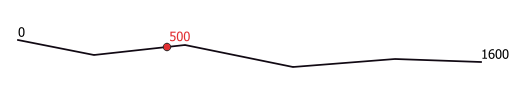Рис. 24.63 Interpolated point at 500m of the beginning of the line

См.также

Points along geometry

Parameters¶

Label

Имя

Тип

Описание

Input layer

INPUT

[vector: line, polygon]

Input line or polygon vector layer

Distance

DISTANCE

Default: 0.0

Distance from the beginning of the line

Interpolated points

OUTPUT

[vector: point]

Default: [Create temporary layer]

Specify the output vector layer. One of:

• Create Temporary Layer (TEMPORARY_OUTPUT)

• Save to File…

• Save to Geopackage…

• Save to PostGIS Table…

The file encoding can also be changed here.

Outputs¶

Label

Имя

Тип

Описание

Interpolated points

OUTPUT

[vector: point]

The output point vector layer with features at a set distance along the line or polygon boundary

Python code¶

Algorithm ID: native:interpolatepoint

import processing
processing.run("algorithm_id", {parameter_dictionary})

The algorithm id is displayed when you hover over the algorithm in the Processing Toolbox. The parameter dictionary provides the parameter NAMEs and values. See Изпользование алгоритмов геообработки в консоли for details on how to run processing algorithms from the Python console.

24.1.16.39. Keep N biggest parts¶

Takes a layer with polygons or multipolygons and returns a new layer in which only the n largest polygons of each multipolygon feature are kept. If a feature has n or fewer parts, the feature will just be copied.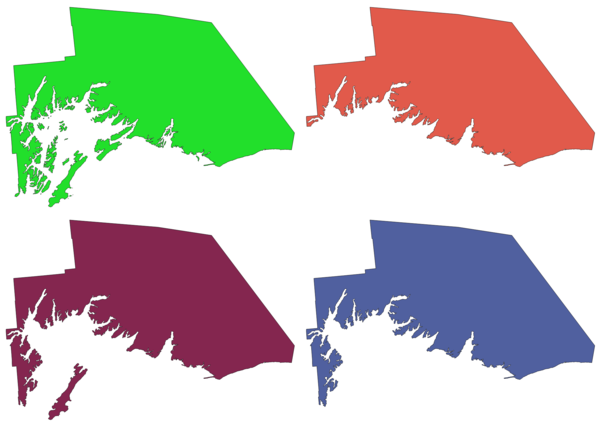Рис. 24.64 Clockwise from top left: original multipart feature, one, two and three biggest parts kept

Parameters¶

Label

Имя

Тип

Описание

Polygons

INPUT

[vector: polygon]

Input polygon vector layer

Parts to keep

PARTS

[number]

Default: 1

Number of parts to keep. If 1, only the biggest part of the feature will be kept.

Parts

OUTPUT

[vector: polygon]

Default: [Create temporary layer]

Specify the output polygon vector layer. One of:

• Create Temporary Layer (TEMPORARY_OUTPUT)

• Save to File…

• Save to Geopackage…

• Save to PostGIS Table…

The file encoding can also be changed here.

Outputs¶

Label

Имя

Тип

Описание

Parts

OUTPUT

[vector: polygon]

The output polygon vector layer with the N biggest parts of each feature

Python code¶

Algorithm ID: qgis:keepnbiggestparts

import processing
processing.run("algorithm_id", {parameter_dictionary})

The algorithm id is displayed when you hover over the algorithm in the Processing Toolbox. The parameter dictionary provides the parameter NAMEs and values. See Изпользование алгоритмов геообработки в консоли for details on how to run processing algorithms from the Python console.

24.1.16.40. Line substring¶

Returns the portion of a line (or curve) which falls between the specified start and end distances (measured from the beginning of the line).

Z and M values are linearly interpolated from existing values.

If a multipart geometry is encountered, only the first part is considered when calculating the substring.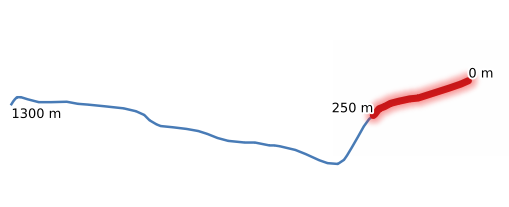Рис. 24.65 Substring line with starting distance set at 0 meters and the ending distance at 250 meters.

См.также

Extend lines

Parameters¶

Label

Имя

Тип

Описание

Input layer

INPUT

[vector: line]

Input line vector layer

Start distance

START_DISTANCE

Distance along the input line to the start point of the output feature

End distance

END_DISTANCE

Distance along the input line to the end point of the output feature

Substring

OUTPUT

[vector: line]

Default: [Create temporary layer]

Specify the output line vector layer. One of:

• Create Temporary Layer (TEMPORARY_OUTPUT)

• Save to File…

• Save to Geopackage…

• Save to PostGIS Table…

The file encoding can also be changed here.

Outputs¶

Label

Имя

Тип

Описание

Substring

OUTPUT

[vector: line]

The output line vector layer.

Python code¶

Algorithm ID: native:linesubstring

import processing
processing.run("algorithm_id", {parameter_dictionary})

The algorithm id is displayed when you hover over the algorithm in the Processing Toolbox. The parameter dictionary provides the parameter NAMEs and values. See Изпользование алгоритмов геообработки в консоли for details on how to run processing algorithms from the Python console.

24.1.16.41. Lines to polygons¶

Generates a polygon layer using as polygon rings the lines from an input line layer.

The attribute table of the output layer is the same as the one of the input layer.

Default menu: Vector ► Geometry Tools

См.также

Parameters¶

Label

Имя

Тип

Описание

Input layer

INPUT

[vector: line]

Input line vector layer

Polygons

OUTPUT

[vector: polygon]

Default: [Create temporary layer]

Specify the output polygon vector layer. One of:

• Create Temporary Layer (TEMPORARY_OUTPUT)

• Save to File…

• Save to Geopackage…

• Save to PostGIS Table…

The file encoding can also be changed here.

Outputs¶

Label

Имя

Тип

Описание

Polygons

OUTPUT

[vector: polygon]

The output polygon vector layer.

Python code¶

Algorithm ID: qgis:linestopolygons

import processing
processing.run("algorithm_id", {parameter_dictionary})

The algorithm id is displayed when you hover over the algorithm in the Processing Toolbox. The parameter dictionary provides the parameter NAMEs and values. See Изпользование алгоритмов геообработки в консоли for details on how to run processing algorithms from the Python console.

24.1.16.42. Merge lines¶

Joins all connected parts of MultiLineString geometries into single LineString geometries.

If any parts of the input MultiLineString geometries are not connected, the resultant geometry will be a MultiLineString containing any lines which could be merged and any non-connected line parts.

Parameters¶

Label

Имя

Тип

Описание

Input layer

INPUT

[vector: line]

Input line vector layer

Merged

OUTPUT

[vector: line]

Default: [Create temporary layer]

Specify the output line vector layer. One of:

• Create Temporary Layer (TEMPORARY_OUTPUT)

• Save to File…

• Save to Geopackage…

• Save to PostGIS Table…

The file encoding can also be changed here.

Outputs¶

Label

Имя

Тип

Описание

Merged

OUTPUT

[vector: line]

The output (merged) line vector layer.

Python code¶

Algorithm ID: native:mergelines

import processing
processing.run("algorithm_id", {parameter_dictionary})

The algorithm id is displayed when you hover over the algorithm in the Processing Toolbox. The parameter dictionary provides the parameter NAMEs and values. See Изпользование алгоритмов геообработки в консоли for details on how to run processing algorithms from the Python console.

24.1.16.43. Minimum bounding geometry¶

Creates geometries which enclose the features from an input layer. The features can be grouped by a field. The output layer will then contain one feature per group value with a geometry (MBB) that covers the geometries of the features with matching value.

The following enclosing geometry types are supported:

• bounding box (envelope)

• oriented rectangle

• circle

• convex hull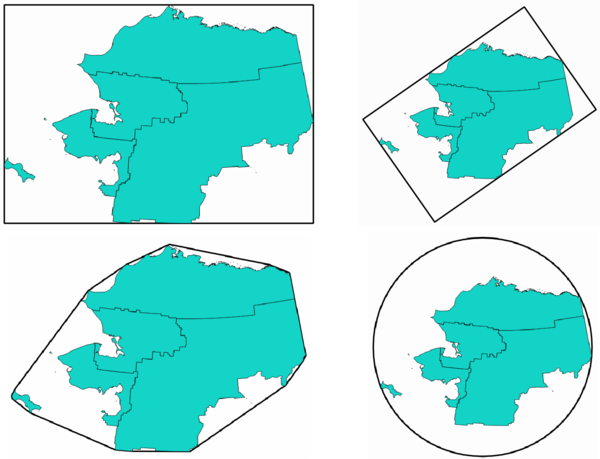Рис. 24.66 Clockwise from top left: envelope, oriented rectangle, circle, convex hull

См.также

Minimum enclosing circles

Parameters¶

Label

Имя

Тип

Описание

Input layer

INPUT

[vector: any]

Input vector layer

Field

Optional

FIELD

[tablefield: any]

Features can be grouped by a field. If set, this causes the output layer to contain one feature per grouped value with a minimal geometry covering only the features with matching values.

Geometry type

TYPE

[enumeration]

Default: 0

Enclosing geometry types. One of:

• 0 — Envelope (Bounding Box)

• 1 — Minimum Oriented Rectangle

• 2 — Minimum Enclosing Circle

• 3 — Convex Hull

Bounding geometry

OUTPUT

[vector: polygon]

Default: [Create temporary layer]

Specify the output polygon vector layer. One of:

• Create Temporary Layer (TEMPORARY_OUTPUT)

• Save to File…

• Save to Geopackage…

• Save to PostGIS Table…

The file encoding can also be changed here.

Outputs¶

Label

Имя

Тип

Описание

Bounding geometry

OUTPUT

[vector: polygon]

The output (bounding) polygon vector layer.

Python code¶

Algorithm ID: qgis:minimumboundinggeometry

import processing
processing.run("algorithm_id", {parameter_dictionary})

The algorithm id is displayed when you hover over the algorithm in the Processing Toolbox. The parameter dictionary provides the parameter NAMEs and values. See Изпользование алгоритмов геообработки в консоли for details on how to run processing algorithms from the Python console.

24.1.16.44. Minimum enclosing circles¶

Calculates the minimum enclosing circles of the features in the input layer.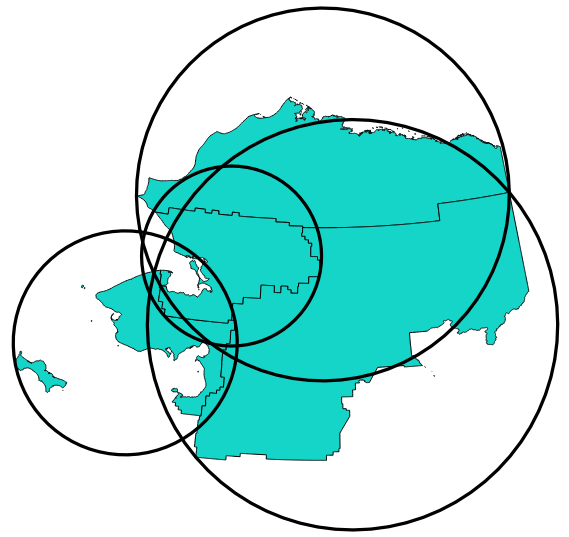Рис. 24.67 Enclosing circles for each feature

См.также

Minimum bounding geometry

Parameters¶

Label

Имя

Тип

Описание

Input layer

INPUT

[vector: any]

Input vector layer

Number of segment in circles

SEGMENTS

[number]

Default: 72

The number of segment used to approximate a circle. Minimum 8, maximum 100000.

Minimum enclosing circles

OUTPUT

[vector: polygon]

Default: [Create temporary layer]

Specify the output polygon vector layer. One of:

• Create Temporary Layer (TEMPORARY_OUTPUT)

• Save to File…

• Save to Geopackage…

• Save to PostGIS Table…

The file encoding can also be changed here.

Outputs¶

Label

Имя

Тип

Описание

Minimum enclosing circles

OUTPUT

[vector: polygon]

The output polygon vector layer.

Python code¶

Algorithm ID: native:minimumenclosingcircle

import processing
processing.run("algorithm_id", {parameter_dictionary})

The algorithm id is displayed when you hover over the algorithm in the Processing Toolbox. The parameter dictionary provides the parameter NAMEs and values. See Изпользование алгоритмов геообработки в консоли for details on how to run processing algorithms from the Python console.

24.1.16.45. Multi-ring buffer (constant distance)¶

Computes multi-ring (donut) buffer for the features of the input layer, using a fixed or dynamic distance and number of rings.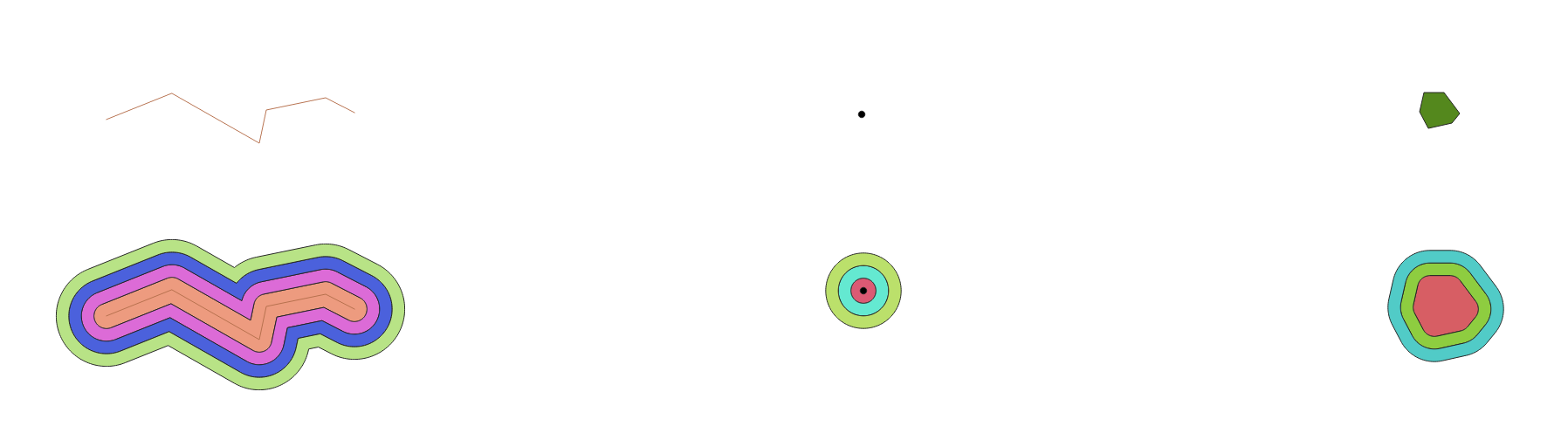Рис. 24.68 Multi-ring buffer for a line, point and polygon layer

Parameters¶

Label

Имя

Тип

Описание

Input layer

INPUT

[vector: any]

Input vector layer

Number of rings

RINGS

Default: 1

The number of rings. It can be a unique value (same number of rings for all the features) or it can be taken from features data (the number of rings depends on feature values).

Distance between rings

DISTANCE

Default: 1.0

Distance between the rings. It can be a unique value (same distance for all the features) or it can be taken from features data (the distance depends on feature values).

Multi-ring buffer (constant distance)

OUTPUT

[vector: polygon]

Default: [Create temporary layer]

Specify the output polygon vector layer. One of:

• Create Temporary Layer (TEMPORARY_OUTPUT)

• Save to File…

• Save to Geopackage…

• Save to PostGIS Table…

The file encoding can also be changed here.

Outputs¶

Label

Имя

Тип

Описание

Multi-ring buffer (constant distance)

OUTPUT

[vector: polygon]

The output polygon vector layer.

Python code¶

Algorithm ID: native:multiringconstantbuffer

import processing
processing.run("algorithm_id", {parameter_dictionary})

The algorithm id is displayed when you hover over the algorithm in the Processing Toolbox. The parameter dictionary provides the parameter NAMEs and values. See Изпользование алгоритмов геообработки в консоли for details on how to run processing algorithms from the Python console.

24.1.16.46. Multipart to singleparts¶

Splits multipart features in the input layer into singlepart features.

The attributes of the output layer are the same as the original ones but divided into single features.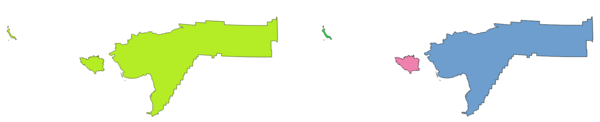Рис. 24.69 Left the multipart source layer and right the single part output result

Default menu: Vector ► Geometry Tools

См.также

Parameters¶

Label

Имя

Тип

Описание

Input layer

INPUT

[vector: any]

Input vector layer

Single parts

OUTPUT

[same as input]

Default: [Create temporary layer]

Specify the output polygon vector layer. One of:

• Create Temporary Layer (TEMPORARY_OUTPUT)

• Save to File…

• Save to Geopackage…

• Save to PostGIS Table…

The file encoding can also be changed here.

Outputs¶

Label

Имя

Тип

Описание

Single parts

OUTPUT

[same as input]

The output vector layer.

Python code¶

Algorithm ID: native:multiparttosingleparts

import processing
processing.run("algorithm_id", {parameter_dictionary})

The algorithm id is displayed when you hover over the algorithm in the Processing Toolbox. The parameter dictionary provides the parameter NAMEs and values. See Изпользование алгоритмов геообработки в консоли for details on how to run processing algorithms from the Python console.

24.1.16.47. Offset lines¶

Offsets lines by a specified distance. Positive distances will offset lines to the left, and negative distances will offset them to the right.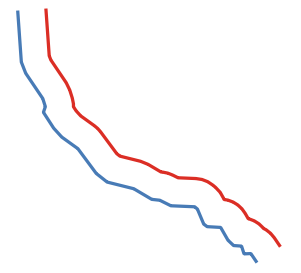Рис. 24.70 In blue the source layer, in red the offset one

Parameters¶

Label

Имя

Тип

Описание

Input layer

INPUT

[vector: line]

Input line vector layer

Distance

DISTANCE

Default: 10.0

Offset distance. You can use the Data Defined button on the right to choose a field from which the radius will be calculated. This way you can have different radius for each feature (see Variable distance buffer).

Segments

SEGMENTS

[number]

Default: 8

Controls the number of line segments to use to approximate a quarter circle when creating rounded offsets.

Join style

JOIN_STYLE

[enumeration]

Default: 0

Specifies whether round, miter or beveled joins should be used when offsetting corners in a line. Options are:

• 0 — Round

• 1 — Miter

• 2 — Bevel

Miter limit

MITER_LIMIT

[number]

Default: 2.0

Controls the maximum distance from the offset curve to use when creating a mitered join (only applicable for miter join styles). Minimum: 1.

Offset

OUTPUT

[vector: line]

Default: [Create temporary layer]

Specify the output (offset) layer. One of:

• Create Temporary Layer (TEMPORARY_OUTPUT)

• Save to File…

• Save to Geopackage…

• Save to PostGIS Table…

The file encoding can also be changed here.

Outputs¶

Label

Имя

Тип

Описание

Offset

OUTPUT

[vector: line]

Output (offset) line layer

Python code¶

Algorithm ID: native:offsetline

import processing
processing.run("algorithm_id", {parameter_dictionary})

The algorithm id is displayed when you hover over the algorithm in the Processing Toolbox. The parameter dictionary provides the parameter NAMEs and values. See Изпользование алгоритмов геообработки в консоли for details on how to run processing algorithms from the Python console.

24.1.16.48. Oriented minimum bounding box¶

Calculates the minimum area rotated rectangle for each feature in the input layer.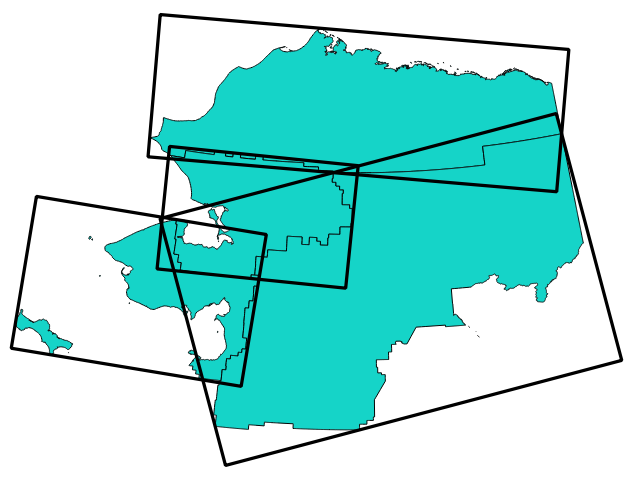Рис. 24.71 Oriented minimum bounding box

См.также

Minimum bounding geometry

Parameters¶

Label

Имя

Тип

Описание

Input layer

INPUT

[vector: any]

Input vector layer

Bounding boxes

OUTPUT

[vector: polygon]

Default: [Create temporary layer]

Specify the output polygon vector layer. One of:

• Create Temporary Layer (TEMPORARY_OUTPUT)

• Save to File…

• Save to Geopackage…

• Save to PostGIS Table…

The file encoding can also be changed here.

Outputs¶

Label

Имя

Тип

Описание

Bounding boxes

OUTPUT

[vector: polygon]

The output polygon vector layer.

Python code¶

Algorithm ID: native:orientedminimumboundingbox

import processing
processing.run("algorithm_id", {parameter_dictionary})

The algorithm id is displayed when you hover over the algorithm in the Processing Toolbox. The parameter dictionary provides the parameter NAMEs and values. See Изпользование алгоритмов геообработки в консоли for details on how to run processing algorithms from the Python console.

24.1.16.49. Orthogonalize¶

Attempts to orthogonalize the geometries of the input line or polygon layer. This process shifts the vertices in the geometries to try to make every angle in the geometry either a right angle or a straight line.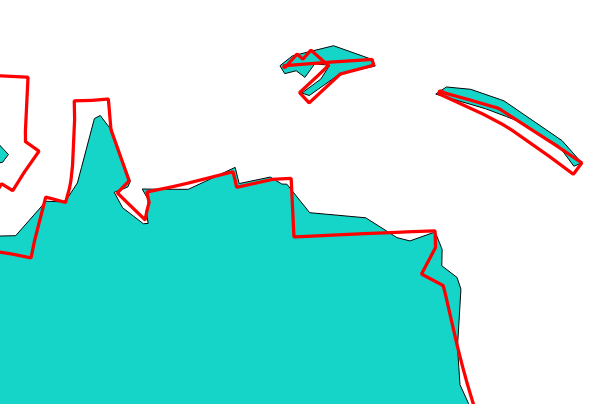Рис. 24.72 In blue the source layer and in the red orthogonalized result

Parameters¶

Label

Имя

Тип

Описание

Input layer

INPUT

[vector: line, polygon]

Input line or polygon vector layer

Maximum angle tolerance (degrees)

ANGLE_TOLERANCE

[number]

Default: 15

Specify the maximum deviation from a right angle or straight line a vertex can have for it to be adjusted. Smaller tolerances mean that only vertices which are already closer to right angles will be adjusted, and larger tolerances mean that vertices which deviate further from right angles will also be adjusted.

Maximum algorithm iterations

MAX_ITERATIONS

[number]

Default: 1000

Setting a larger number for the maximum number of iterations will result in a more orthogonal geometry at the cost of extra processing time.

Orthogonalized

OUTPUT

[same as input]

Default: [Create temporary layer]

Specify the output polygon vector layer. One of:

• Create Temporary Layer (TEMPORARY_OUTPUT)

• Save to File…

• Save to Geopackage…

• Save to PostGIS Table…

The file encoding can also be changed here.

Outputs¶

Label

Имя

Тип

Описание

Orthogonalized

OUTPUT

[same as input]

The output polygon vector layer with adjusted angles.

Python code¶

Algorithm ID: native:orthogonalize

import processing
processing.run("algorithm_id", {parameter_dictionary})

The algorithm id is displayed when you hover over the algorithm in the Processing Toolbox. The parameter dictionary provides the parameter NAMEs and values. See Изпользование алгоритмов геообработки в консоли for details on how to run processing algorithms from the Python console.

24.1.16.50. Point on Surface¶

For each feature of the input layer, returns a point that is guaranteed to lie on the surface of the feature geometry.

См.также

Centroids

Parameters¶

Label

Имя

Тип

Описание

Input layer

INPUT

[vector: any]

Input vector layer

Create point on surface for each part

ANGLE_TOLERANCE

If checked, a point will be created for each part of the geometry.

Point

OUTPUT

[vector: point]

Default: [Create temporary layer]

Specify the output point vector layer. One of:

• Create Temporary Layer (TEMPORARY_OUTPUT)

• Save to File…

• Save to Geopackage…

• Save to PostGIS Table…

The file encoding can also be changed here.

Outputs¶

Label

Имя

Тип

Описание

Point

OUTPUT

[vector: point]

The output point vector layer.

Python code¶

Algorithm ID: native:pointonsurface

import processing
processing.run("algorithm_id", {parameter_dictionary})

The algorithm id is displayed when you hover over the algorithm in the Processing Toolbox. The parameter dictionary provides the parameter NAMEs and values. See Изпользование алгоритмов геообработки в консоли for details on how to run processing algorithms from the Python console.

24.1.16.51. Points along geometry¶

Creates points at regular intervals along line or polygon geometries. Created points will have new attributes added for the distance along the geometry and the angle of the line at the point.

An optional start and end offset can be specified, which controls how far from the start and end of the geometry the points should be created.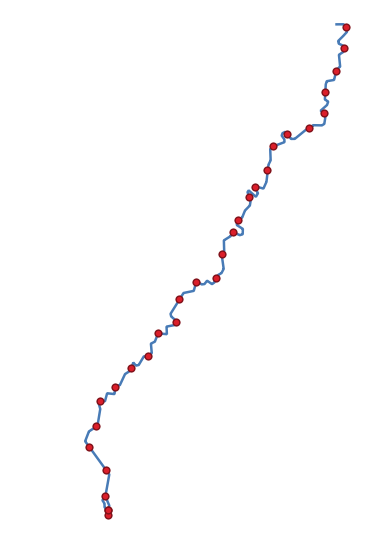Рис. 24.73 Points created along the source line layer

См.также

Interpolate point on line

Parameters¶

Label

Имя

Тип

Описание

Input layer

INPUT

[vector: line, polygon]

Input line or polygon vector layer

Distance

DISTANCE

Default: 1.0

Distance between two consecutive points along the line

Start offset

START_OFFSET

Default: 0.0

Distance from the beginning of the input line, representing the position of the first point.

End offset

END_OFFSET

Default: 0.0

Distance from the end of the input line, representing the position beyond which no point feature shoud be created.

Interpolated points

OUTPUT

[vector: point]

Default: [Create temporary layer]

Specify the output vector layer. One of:

• Create Temporary Layer (TEMPORARY_OUTPUT)

• Save to File…

• Save to Geopackage…

• Save to PostGIS Table…

The file encoding can also be changed here.

Outputs¶

Label

Имя

Тип

Описание

Interpolated points

OUTPUT

[vector: point]

Point vector layer with features placed along lines or polygon boundaries of the input layer.

Python code¶

Algorithm ID: native:pointsalonglines

import processing
processing.run("algorithm_id", {parameter_dictionary})

The algorithm id is displayed when you hover over the algorithm in the Processing Toolbox. The parameter dictionary provides the parameter NAMEs and values. See Изпользование алгоритмов геообработки в консоли for details on how to run processing algorithms from the Python console.

24.1.16.52. Points displacement¶

Given a distance of proximity, identifies nearby point features and radially distributes them over a circle whose center represents their barycenter. A convenient tool to scatter overlaid features.

Parameters¶

Label

Имя

Тип

Описание

Input layer

INPUT

[vector: point]

Input point vector layer

Minimum distance to other points

PROXIMITY

[number]

Default: 1.0

Distance below which point features are considered close. Close features are distributed altogether.

Displacement distance

DISTANCE

[number]

Default: 1.0

Radius of the circle on which close features are placed

Horizontal distribution for two point case

HORIZONTAL

[boolean]

Default: False

When only two points are identified as close, aligns them horizontally on the circle instead of vertically.

Displaced

OUTPUT

[vector: point]

Default: [Create temporary layer]

Specify the output vector layer. One of:

• Create Temporary Layer (TEMPORARY_OUTPUT)

• Save to File…

• Save to Geopackage…

• Save to PostGIS Table…

The file encoding can also be changed here.

Outputs¶

Label

Имя

Тип

Описание

Displaced

OUTPUT

[vector: point]

Output point vector layer

Python code¶

Algorithm ID: qgis:pointsdisplacement

import processing
processing.run("algorithm_id", {parameter_dictionary})

The algorithm id is displayed when you hover over the algorithm in the Processing Toolbox. The parameter dictionary provides the parameter NAMEs and values. See Изпользование алгоритмов геообработки в консоли for details on how to run processing algorithms from the Python console.

24.1.16.53. Pole of inaccessibility¶

Calculates the pole of inaccessibility for a polygon layer, which is the most distant internal point from the boundary of the surface.

This algorithm uses the „polylabel“ algorithm (Vladimir Agafonkin, 2016), which is an iterative approach guaranteed to find the true pole of inaccessibility within a specified tolerance. A more precise tolerance (lower value) requires more iterations and will take longer to calculate.

The distance from the calculated pole to the polygon boundary will be stored as a new attribute in the output layer.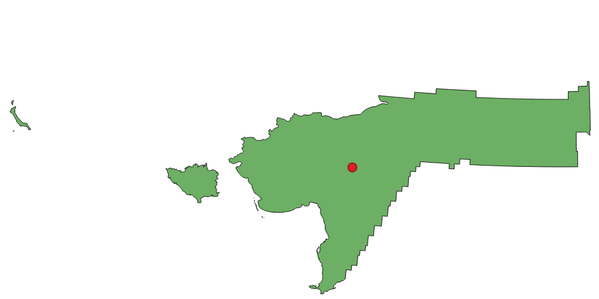Рис. 24.74 Pole of inaccessibility

Parameters¶

Label

Имя

Тип

Описание

Input layer

INPUT

[vector: polygon]

Input vector layer

Tolerance

TOLERANCE

[number]

Default: 1.0

Set the tolerance for the calculation

Point

OUTPUT

[vector: point]

Default: [Create temporary layer]

Specify the output polygon vector layer. One of:

• Create Temporary Layer (TEMPORARY_OUTPUT)

• Save to File…

• Save to Geopackage…

• Save to PostGIS Table…

The file encoding can also be changed here.

Outputs¶

Label

Имя

Тип

Описание

Point

OUTPUT

[vector: point]

The output point vector layer

Python code¶

Algorithm ID: native:poleofinaccessibility

import processing
processing.run("algorithm_id", {parameter_dictionary})

The algorithm id is displayed when you hover over the algorithm in the Processing Toolbox. The parameter dictionary provides the parameter NAMEs and values. See Изпользование алгоритмов геообработки в консоли for details on how to run processing algorithms from the Python console.

24.1.16.54. Polygonize¶

Creates a polygon layer whose features boundaries are generated from a line layer of closed features.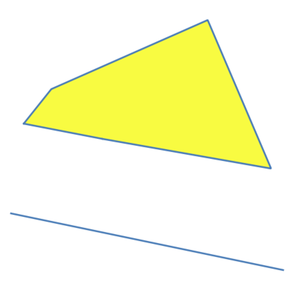Рис. 24.75 The yellow polygons generated from the closed lines

Примечание

The line layer must have closed shapes in order to be transformed into a polygon.

Parameters¶

Label

Имя

Тип

Описание

Input layer

INPUT

[vector: line]

Input line vector layer

Keep table structure of line layer

Optional

KEEP_FIELDS

[boolean]

Default: False

Check to keep the fields (only the table structure, not the values) of the input layer

Polygons from lines

OUTPUT

[vector: polygon]

Default: [Create temporary layer]

Specify the output polygon vector layer. One of:

• Create Temporary Layer (TEMPORARY_OUTPUT)

• Save to File…

• Save to Geopackage…

• Save to PostGIS Table…

The file encoding can also be changed here.

Outputs¶

Label

Имя

Тип

Описание

Polygons from lines

OUTPUT

[vector: polygon]

The output polygon vector layer from lines

Python code¶

Algorithm ID: native:polygonize

import processing
processing.run("algorithm_id", {parameter_dictionary})

The algorithm id is displayed when you hover over the algorithm in the Processing Toolbox. The parameter dictionary provides the parameter NAMEs and values. See Изпользование алгоритмов геообработки в консоли for details on how to run processing algorithms from the Python console.

24.1.16.55. Polygons to lines¶

Takes a polygon layer and creates a line layer, with lines representing the boundaries of the polygons in the input layer.

The attribute table of the output layer is the same as the one of the input layer.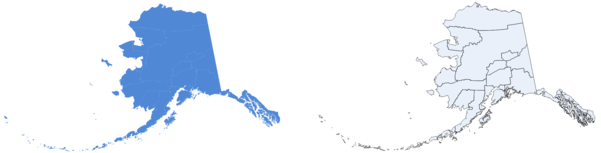Рис. 24.76 Black lines as the result of the algorithm

Default menu: Vector ► Geometry Tools

См.также

Parameters¶

Label

Имя

Тип

Описание

Input layer

INPUT

[vector: polygon]

Input polygon vector layer

Lines

OUTPUT

[vector: line]

Default: [Create temporary layer]

Specify the output line vector layer. One of:

• Create Temporary Layer (TEMPORARY_OUTPUT)

• Save to File…

• Save to Geopackage…

• Save to PostGIS Table…

The file encoding can also be changed here.

Outputs¶

Label

Имя

Тип

Описание

Lines

OUTPUT

[vector: line]

The output line vector layer from polygons

Python code¶

Algorithm ID: native:polygonstolines

import processing
processing.run("algorithm_id", {parameter_dictionary})

The algorithm id is displayed when you hover over the algorithm in the Processing Toolbox. The parameter dictionary provides the parameter NAMEs and values. See Изпользование алгоритмов геообработки в консоли for details on how to run processing algorithms from the Python console.

24.1.16.56. Project points (Cartesian)¶

Projects point geometries by a specified distance and bearing (azimuth).

Parameters¶

Label

Имя

Тип

Описание

Input layer

INPUT

[vector: point]

Input point vector layer

Bearing (degrees from North)

BEARING

Default: 0.0

Clockwise angle starting from North, in degree (°) unit

Distance

DISTANCE

Default: 1.0

Distance to offset geometries, in layer units

Projected

OUTPUT

[vector: point]

Default: [Create temporary layer]

Specify the output point vector layer. One of:

• Create Temporary Layer (TEMPORARY_OUTPUT)

• Save to File…

• Save to Geopackage…

• Save to PostGIS Table…

The file encoding can also be changed here.

Outputs¶

Label

Имя

Тип

Описание

Projected

OUTPUT

[vector: point]

The output (projected) point vector layer

Python code¶

Algorithm ID: native:projectpointcartesian

import processing
processing.run("algorithm_id", {parameter_dictionary})

The algorithm id is displayed when you hover over the algorithm in the Processing Toolbox. The parameter dictionary provides the parameter NAMEs and values. See Изпользование алгоритмов геообработки в консоли for details on how to run processing algorithms from the Python console.

24.1.16.57. Promote to multipart¶

Takes a vector layer with singlepart geometries and generates a new one in which all geometries are multipart.

Input features which are already multipart features will remain unchanged.

This algorithm can be used to force geometries to multipart types in order to be compatible with data providers that require multipart features.

См.также

Parameters¶

Label

Имя

Тип

Описание

Input layer

INPUT

[vector: any]

Input vector layer

Multiparts

OUTPUT

[same as input]

Default: [Create temporary layer]

Specify the output multipart vector layer. One of:

• Create Temporary Layer (TEMPORARY_OUTPUT)

• Save to File…

• Save to Geopackage…

• Save to PostGIS Table…

The file encoding can also be changed here.

Outputs¶

Label

Имя

Тип

Описание

Multiparts

OUTPUT

[same as input]

The output multipart vector layer

Python code¶

Algorithm ID: native:promotetomulti

import processing
processing.run("algorithm_id", {parameter_dictionary})

The algorithm id is displayed when you hover over the algorithm in the Processing Toolbox. The parameter dictionary provides the parameter NAMEs and values. See Изпользование алгоритмов геообработки в консоли for details on how to run processing algorithms from the Python console.

24.1.16.58. Rectangles, ovals, diamonds¶

Creates a buffer area with a rectangle, oval or diamond shape for each feature of the input point layer.

The shape parameters can be fixed for all features or dynamic using a field or an expression.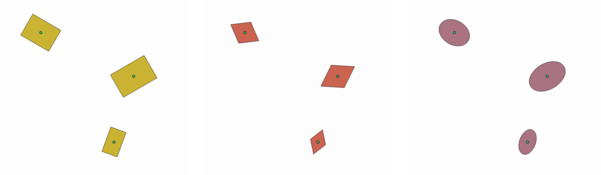Рис. 24.77 Different buffer shapes with dynamic parameters

Parameters¶

Label

Имя

Тип

Описание

Input layer

INPUT

[vector: point]

Input point vector layer

Buffer shape

SHAPE

[enumeration]

The shape to use. One of:

• 0 — Rectangles

• 1 — Ovals

• 2 — Diamonds

Width

WIDTH

Default: 1.0

Width of the buffer shape

Height

HEIGHT

Default: 1.0

Height of the buffer shape

Rotation

Optional

ROTATION

Default: None

Rotation of the buffer shape

Number of segment

SEGMENTS

[number]

Default: 36

Number of segments for a full circle (Ovals shape)

Вывод

OUTPUT

[vector: polygon]

Default: [Create temporary layer]

Specify the output vector layer. One of:

• Create Temporary Layer (TEMPORARY_OUTPUT)

• Save to File…

• Save to Geopackage…

• Save to PostGIS Table…

The file encoding can also be changed here.

Outputs¶

Label

Имя

Тип

Описание

Вывод

OUTPUT

[vector: polygon]

The output vector layer (with the buffer shapes)

Python code¶

Algorithm ID: native:rectanglesovalsdiamonds

import processing
processing.run("algorithm_id", {parameter_dictionary})

The algorithm id is displayed when you hover over the algorithm in the Processing Toolbox. The parameter dictionary provides the parameter NAMEs and values. See Изпользование алгоритмов геообработки в консоли for details on how to run processing algorithms from the Python console.

24.1.16.59. Remove duplicate vertices¶

Removes duplicate vertices from features, wherever removing the vertices does not result in a degenerate geometry.

The tolerance parameter specifies the tolerance for coordinates when determining whether vertices are identical.

By default, Z values are not considered when detecting duplicate vertices. E.g. two vertices with the same X and Y coordinate but different Z values will still be considered duplicate and one will be removed. If the Use Z Value parameter is true, then the Z values are also tested and vertices with the same X and Y but different Z will be maintained.

Примечание

Duplicate vertices are not tested between different parts of a multipart geometry, e.g. a multipoint geometry with overlapping points will not be changed by this method.

Parameters¶

Label

Имя

Тип

Описание

Input layer

INPUT

[vector: any]

Input vector layer

Tolerance

TOLERANCE

Default: 0.000001

Vertices closer than the specified distance are considered duplicates

Use Z value

USE_Z_VALUE

Default: False

If the Use Z Value parameter is true, then the Z values are also tested and vertices with the same X and Y but different Z will be maintained.

Cleaned

OUTPUT

[same as input]

Default: [Create temporary layer]

Specify the output vector layer. One of:

• Create Temporary Layer (TEMPORARY_OUTPUT)

• Save to File…

• Save to Geopackage…

• Save to PostGIS Table…

The file encoding can also be changed here.

Outputs¶

Label

Имя

Тип

Описание

Cleaned

OUTPUT

[same as input]

The output vector layer (without duplicate vertices)

Python code¶

Algorithm ID: native:removeduplicatevertices

import processing
processing.run("algorithm_id", {parameter_dictionary})

The algorithm id is displayed when you hover over the algorithm in the Processing Toolbox. The parameter dictionary provides the parameter NAMEs and values. See Изпользование алгоритмов геообработки в консоли for details on how to run processing algorithms from the Python console.

24.1.16.60. Remove null geometries¶

Removes any features which do not have a geometry from a vector layer. All other features will be copied unchanged.

The features with null geometries can be saved to a separate layer.

If Also remove empty geometries is checked, the algorithm removes features whose geometries have no coordinates, i.e., geometries that are empty. In that case, also the null output will reflect this option, containing both null and empty geometries.

См.также

Delete duplicate geometries

Parameters¶

Label

Имя

Тип

Описание

Input layer

INPUT

[vector: any]

Input vector layer (with non-NULL geometries)

Also remove empty geometries

REMOVE_EMPTY

[boolean]

Non null geometries

OUTPUT

[same as input]

Default: [Create temporary layer]

Specify the output vector layer for the non-NULL (and non-empty) geometries. One of:

• Create Temporary Layer (TEMPORARY_OUTPUT)

• Save to File…

• Save to Geopackage…

• Save to PostGIS Table…

The file encoding can also be changed here.

Null geometries

NULL_OUTPUT

[same as input]

Default: [Skip output]

Specify the output vector layer for the NULL (and empty) geometries. One of:

• Skip Output

• Create Temporary Layer (TEMPORARY_OUTPUT)

• Save to File…

• Save to Geopackage…

• Save to PostGIS Table…

The file encoding can also be changed here.

Outputs¶

Label

Имя

Тип

Описание

Null geometries

NULL_OUTPUT

[same as input]

Output vector layer (for NULL and, if chosen, empty geometries)

Non null geometries

OUTPUT

[same as input]

The output vector layer (without NULL and, if chosen, empty geometries)

Python code¶

Algorithm ID: native:removenullgeometries

import processing
processing.run("algorithm_id", {parameter_dictionary})

The algorithm id is displayed when you hover over the algorithm in the Processing Toolbox. The parameter dictionary provides the parameter NAMEs and values. See Изпользование алгоритмов геообработки в консоли for details on how to run processing algorithms from the Python console.

24.1.16.61. Reverse line direction¶

Inverts the direction of a line layer.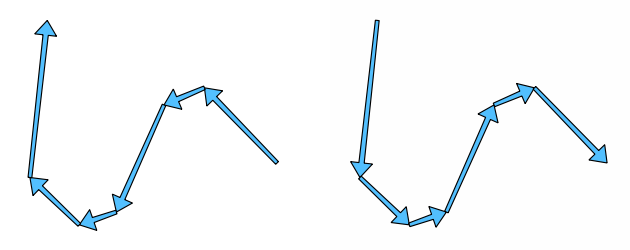Рис. 24.78 Before and after the direction inversion

Parameters¶

Label

Имя

Тип

Описание

Input layer

INPUT

[vector: line]

Input line vector layer

Reversed

OUTPUT

[vector: line]

Default: [Create temporary layer]

Specify the output line vector layer. One of:

• Create Temporary Layer (TEMPORARY_OUTPUT)

• Save to File…

• Save to Geopackage…

• Save to PostGIS Table…

The file encoding can also be changed here.

Outputs¶

Label

Имя

Тип

Описание

Reversed

OUTPUT

[vector: line]

The output line vector layer (with reversed lines)

Python code¶

Algorithm ID: native:reverselinedirection

import processing
processing.run("algorithm_id", {parameter_dictionary})

The algorithm id is displayed when you hover over the algorithm in the Processing Toolbox. The parameter dictionary provides the parameter NAMEs and values. See Изпользование алгоритмов геообработки в консоли for details on how to run processing algorithms from the Python console.

24.1.16.62. Rotate¶

Rotates feature geometries by the specified angle clockwise. The rotation occurs around each feature’s centroid, or optionally around a unique preset point.

См.также

Parameters¶

Label

Имя

Тип

Описание

Input layer

INPUT

[vector: any]

Input vector layer

Rotation (degrees clockwise)

ANGLE

Default: 0.0

Angle of the rotation in degrees

Rotation anchor point (x, y)

Optional

ANCHOR

[point]

Default: None

X,Y coordinates of the point to rotate the features around. If not set the rotation occurs around each feature’s centroid.

Rotated

OUTPUT

[same as input]

Default: [Create temporary layer]

Specify the output vector layer (with rotated geometries). One of:

• Create Temporary Layer (TEMPORARY_OUTPUT)

• Save to File…

• Save to Geopackage…

• Save to PostGIS Table…

The file encoding can also be changed here.

Outputs¶

Label

Имя

Тип

Описание

Rotated

OUTPUT

[same as input]

The output vector layer with rotated geometries

Python code¶

Algorithm ID: native:rotatefeatures

import processing
processing.run("algorithm_id", {parameter_dictionary})

The algorithm id is displayed when you hover over the algorithm in the Processing Toolbox. The parameter dictionary provides the parameter NAMEs and values. See Изпользование алгоритмов геообработки в консоли for details on how to run processing algorithms from the Python console.

24.1.16.63. Segmentize by maximum angle¶

Segmentizes a geometry by converting curved sections to linear sections.

The segmentization is performed by specifying the maximum allowed radius angle between vertices on the straightened geometry (e.g the angle of the arc created from the original arc center to consecutive output vertices on the linearized geometry). Non-curved geometries will be retained without change.

См.также

Parameters¶

Label

Имя

Тип

Описание

Input layer

INPUT

[vector: line, polygon]

Input line or polygon vector layer

Maximum angle between vertices (degrees)

ANGLE

Default: 5.0

Maximum allowed radius angle between vertices on the straightened geometry

Segmentized

OUTPUT

[same as input]

Default: [Create temporary layer]

Specify the output vector layer (with segmentized geometries). One of:

• Create Temporary Layer (TEMPORARY_OUTPUT)

• Save to File…

• Save to Geopackage…

• Save to PostGIS Table…

The file encoding can also be changed here.

Outputs¶

Label

Имя

Тип

Описание

Segmentized

OUTPUT

[same as input]

The output vector layer with segmentized geometries

Python code¶

Algorithm ID: native:segmentizebymaxangle

import processing
processing.run("algorithm_id", {parameter_dictionary})

The algorithm id is displayed when you hover over the algorithm in the Processing Toolbox. The parameter dictionary provides the parameter NAMEs and values. See Изпользование алгоритмов геообработки в консоли for details on how to run processing algorithms from the Python console.

24.1.16.64. Segmentize by maximum distance¶

Segmentizes a geometry by converting curved sections to linear sections.

The segmentization is performed by specifying the maximum allowed offset distance between the original curve and the segmentized representation. Non-curved geometries will be retained without change.

См.также

Parameters¶

Label

Имя

Тип

Описание

Input layer

INPUT

[vector: line, polygon]

Input line or polygon vector layer

Maximum offset distance

DISTANCE

Default: 1.0

Maximum allowed offset distance between the original curve and the segmentized representation, in the layer units.

Segmentized

OUTPUT

[same as input]

Default: [Create temporary layer]

Specify the output vector layer (with segmentized geometries). One of:

• Create Temporary Layer (TEMPORARY_OUTPUT)

• Save to File…

• Save to Geopackage…

• Save to PostGIS Table…

The file encoding can also be changed here.

Outputs¶

Label

Имя

Тип

Описание

Segmentized

OUTPUT

[same as input]

The output vector layer with segmentized geometries

Python code¶

Algorithm ID: native:segmentizebymaxdistance

import processing
processing.run("algorithm_id", {parameter_dictionary})

The algorithm id is displayed when you hover over the algorithm in the Processing Toolbox. The parameter dictionary provides the parameter NAMEs and values. See Изпользование алгоритмов геообработки в консоли for details on how to run processing algorithms from the Python console.

24.1.16.65. Set M value¶

Sets the M value for geometries in a layer.

If M values already exist in the layer, they will be overwritten with the new value. If no M values exist, the geometry will be upgraded to include M values and the specified value used as the initial M value for all geometries.

Совет

Use the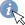Identify Features button to check the added M value: the results are available in the Identify Results dialog.

Parameters¶

Label

Имя

Тип

Описание

Input layer

INPUT

[vector: any]

Input vector layer

M Value

M_VALUE

Default: 0.0

M value to assign to the feature geometries

OUTPUT

[same as input]

Default: [Create temporary layer]

Specify the output vector layer. One of:

• Create Temporary Layer (TEMPORARY_OUTPUT)

• Save to File…

• Save to Geopackage…

• Save to PostGIS Table…

The file encoding can also be changed here.

Outputs¶

Label

Имя

Тип

Описание

OUTPUT

[same as input]

The output vector layer (with M values assigned to the geometries)

Python code¶

Algorithm ID: native:setmvalue

import processing
processing.run("algorithm_id", {parameter_dictionary})

The algorithm id is displayed when you hover over the algorithm in the Processing Toolbox. The parameter dictionary provides the parameter NAMEs and values. See Изпользование алгоритмов геообработки в консоли for details on how to run processing algorithms from the Python console.

24.1.16.66. Set M value from raster¶

Uses values sampled from a band within a raster layer to set the M value for every overlapping vertex in the feature geometry. The raster values can optionally be scaled by a preset amount.

If M values already exist in the layer, they will be overwritten with the new value. If no M values exist, the geometry will be upgraded to include M values.

Parameters¶

Label

Имя

Тип

Описание

Input layer

INPUT

[vector: any]

Input vector layer

Raster layer

RASTER

[raster]

Raster layer with M values

Band number

BAND

[raster band]

Default: 1

The raster band from which the M values are taken

Value for nodata or non-intersecting vertices

NODATA

[number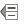] Default: 0.0

Value to use in case the vertex does not intersect (a valid pixel of) the raster

Scale factor

SCALE

Default: 1.0

Scaling value: the band values are multiplied by this value.

Updated

OUTPUT

[same as input]

Default: [Create temporary layer]

Specify the output vector layer (with updated M values). One of:

• Create Temporary Layer (TEMPORARY_OUTPUT)

• Save to File…

• Save to Geopackage…

• Save to PostGIS Table…

The file encoding can also be changed here.

Outputs¶

Label

Имя

Тип

Описание

Updated

OUTPUT

[same as input]

The output vector layer (with updated M values)

Python code¶

Algorithm ID: native:setmfromraster

import processing
processing.run("algorithm_id", {parameter_dictionary})

The algorithm id is displayed when you hover over the algorithm in the Processing Toolbox. The parameter dictionary provides the parameter NAMEs and values. See Изпользование алгоритмов геообработки в консоли for details on how to run processing algorithms from the Python console.

24.1.16.67. Set Z value¶

Sets the Z value for geometries in a layer.

If Z values already exist in the layer, they will be overwritten with the new value. If no Z values exist, the geometry will be upgraded to include Z values and the specified value used as the initial Z value for all geometries.

Совет

Use theIdentify Features button to check the added Z value: the results are available in the Identify Results dialog.

Parameters¶

Label

Имя

Тип

Описание

Input layer

INPUT

[vector: any]

Input vector layer

Z Value

Z_VALUE

Default: 0.0

Z value to assign to the feature geometries

OUTPUT

[same as input]

Default: [Create temporary layer]

Specify the output vector layer. One of:

• Create Temporary Layer (TEMPORARY_OUTPUT)

• Save to File…

• Save to Geopackage…

• Save to PostGIS Table…

The file encoding can also be changed here.

Outputs¶

Label

Имя

Тип

Описание

OUTPUT

[same as input]

The output vector layer (with Z values assigned)

Python code¶

Algorithm ID: native:setzvalue

import processing
processing.run("algorithm_id", {parameter_dictionary})

The algorithm id is displayed when you hover over the algorithm in the Processing Toolbox. The parameter dictionary provides the parameter NAMEs and values. See Изпользование алгоритмов геообработки в консоли for details on how to run processing algorithms from the Python console.

24.1.16.68. Simplify¶

Simplifies the geometries in a line or polygon layer. It creates a new layer with the same features as the ones in the input layer, but with geometries containing a lower number of vertices.

The algorithm gives a choice of simplification methods, including distance based (the «Douglas-Peucker» algorithm), area based («Visvalingam» algorithm) and snapping geometries to grid.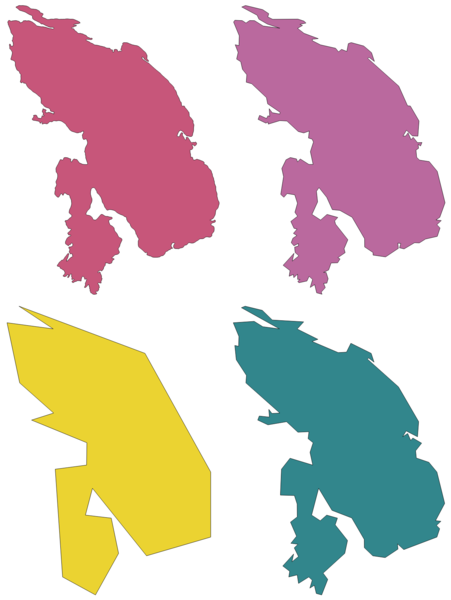Рис. 24.79 Clockwise from top left: source layer and increasing simplification tolerances

Default menu: Vector ► Geometry Tools

См.также

Parameters¶

Label

Имя

Тип

Описание

Input layer

INPUT

[vector: line, polygon]

Input line or polygon vector layer

Simplification method

METHOD

[enumeration]

Default: 0

Simplification method. One of:

• 0 — Distance (Douglas-Peucker)

• 1 — Snap to grid

• 2 — Area (Visvalingam)

Tolerance

TOLERANCE

Default: 1.0

Threshold tolerance (in units of the layer): if the distance between two nodes is smaller than the tolerance value, the segment will be simplified and vertices will be removed.

Simplified

OUTPUT

[same as input]

Default: [Create temporary layer]

Specify the output (simplified) vector layer. One of:

• Create Temporary Layer (TEMPORARY_OUTPUT)

• Save to File…

• Save to Geopackage…

• Save to PostGIS Table…

The file encoding can also be changed here.

Outputs¶

Label

Имя

Тип

Описание

Simplified

OUTPUT

[same as input]

The output (simplified) vector layer

Python code¶

Algorithm ID: native:simplifygeometries

import processing
processing.run("algorithm_id", {parameter_dictionary})

The algorithm id is displayed when you hover over the algorithm in the Processing Toolbox. The parameter dictionary provides the parameter NAMEs and values. See Изпользование алгоритмов геообработки в консоли for details on how to run processing algorithms from the Python console.

24.1.16.69. Single sided buffer¶

Computes a buffer on lines by a specified distance on one side of the line only.

Buffer always results in a polygon layer.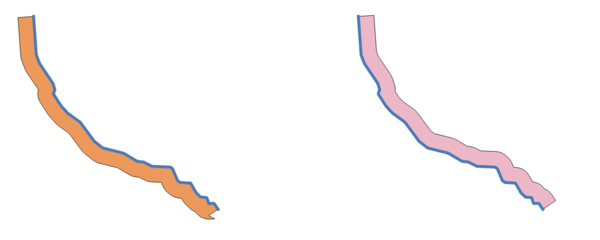Рис. 24.80 Left versus right side buffer on the same vector line layer

См.также

Buffer

Parameters¶

Label

Имя

Тип

Описание

Input layer

INPUT

[vector: line]

Input line vector layer

Distance

DISTANCE

[number]

Default: 10.0

Buffer distance.

Side

SIDE

[enumeration]

Which side to create the buffer on. One of:

• 0 – Left

• 1 – Right

Segments

SEGMENTS

[number]

Default: 8

Controls the number of line segments to use to approximate a quarter circle when creating rounded offsets.

Join style

JOIN_STYLE

[enumeration]

Specifies whether round, miter or beveled joins should be used when offsetting corners in a line. Options are:

• 0 — Round

• 1 — Miter

• 2 — Bevel

Miter limit

MITER_LIMIT

[number]

Default: 2.0

Controls the maximum distance from the offset curve to use when creating a mitered join (only applicable for miter join styles). Minimum: 1.0

Buffer

OUTPUT

[vector: polygon]

Default: [Create temporary layer]

Specify the output (buffer) layer. One of:

• Create Temporary Layer (TEMPORARY_OUTPUT)

• Save to File…

• Save to Geopackage…

• Save to PostGIS Table…

The file encoding can also be changed here.

Outputs¶

Label

Имя

Тип

Описание

Buffer

OUTPUT

[vector: polygon]

Output (buffer) polygon layer

Python code¶

Algorithm ID: native:singlesidedbuffer

import processing
processing.run("algorithm_id", {parameter_dictionary})

The algorithm id is displayed when you hover over the algorithm in the Processing Toolbox. The parameter dictionary provides the parameter NAMEs and values. See Изпользование алгоритмов геообработки в консоли for details on how to run processing algorithms from the Python console.

24.1.16.70. Smooth¶

Smooths the geometries in a line or polygon layer by adding more vertices and corners to the feature geometries.

The iterations parameter dictates how many smoothing iterations will be applied to each geometry. A higher number of iterations results in smoother geometries with the cost of greater number of nodes in the geometries.Рис. 24.81 Increasing number of iterations causes smoother geometries

The offset parameter controls how «tightly» the smoothed geometries follow the original geometries. Smaller values results in a tighter fit, and larger values will create a looser fit.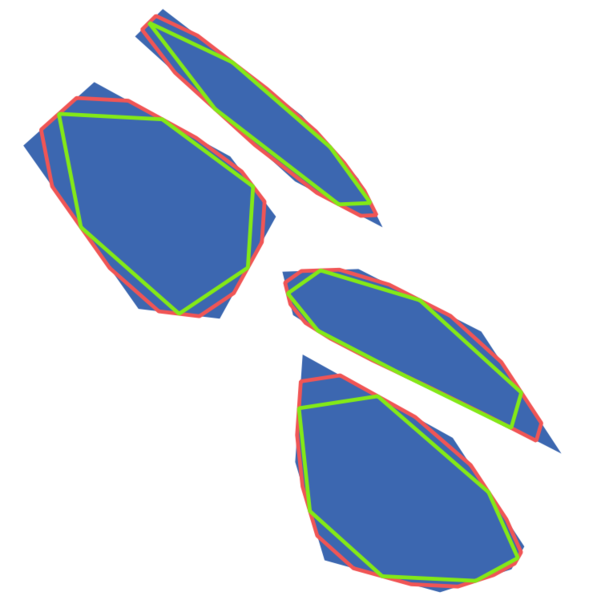Рис. 24.82 Blue: the input layer. Offset 0.25 gives the red line, while offset 0.50 gives the green line.

The maximum angle parameter can be used to prevent smoothing of nodes with large angles. Any node where the angle of the segments to either side is larger than this will not be smoothed. For example, setting the maximum angle to 90 degrees or lower would preserve right angles in the geometry.

См.также

Parameters¶

Label

Имя

Тип

Описание

Input layer

INPUT

[vector: line, polygon]

Input line or polygon vector layer

Iterations

ITERATIONS

Default: 1

Increasing the number of iterations will give smoother geometries (and more vertices).

Offset

OFFSET

Default: 0.25

Increasing values will move the smoothed lines / boundaries further away from the input lines / boundaries.

Maximum node angle to smooth

MAX_ANGLE

Default: 180.0

Every node below this value will be smoothed

Smoothed

OUTPUT

[same as input]

Default: [Create temporary layer]

Specify the output (smoothed) layer. One of:

• Create Temporary Layer (TEMPORARY_OUTPUT)

• Save to File…

• Save to Geopackage…

• Save to PostGIS Table…

The file encoding can also be changed here.

Outputs¶

Label

Имя

Тип

Описание

Smoothed

OUTPUT

[same as input]

Output (smoothed) vector layer

Python code¶

Algorithm ID: native:smoothgeometry

import processing
processing.run("algorithm_id", {parameter_dictionary})

The algorithm id is displayed when you hover over the algorithm in the Processing Toolbox. The parameter dictionary provides the parameter NAMEs and values. See Изпользование алгоритмов геообработки в консоли for details on how to run processing algorithms from the Python console.

24.1.16.71. Snap geometries to layer¶

Snaps the geometries in a layer either to the geometries from another layer, or to geometries within the same layer.

Matching is done based on a tolerance distance, and vertices will be inserted or removed as required to make the geometries match the reference geometries.

См.также

Snap points to grid

Parameters¶

Label

Имя

Тип

Описание

Input layer

INPUT

[vector: any]

Input vector layer

Reference layer

REFERENCE_LAYER

[vector: any]

Vector layer to snap to

Tolerance

TOLERANCE

[number]

Default: 10.0

Control how close input vertices need to be to the reference layer geometries before they are snapped.

Behavior

BEHAVIOR

[enumeration]

Default: 0

Snapping can be done to an existing node or a segment (its closest point to the vertex to move). Available snapping options:

• 0 — Prefer aligning nodes, insert extra vertices where required

Prefer to snap to nodes, even when a segment may be closer than a node. New nodes will be inserted to make geometries follow each other exactly when inside allowable tolerance.

• 1 — Prefer closest point, insert extra vertices where required

Snap to closest point, regardless of it is a node or a segment. New nodes will be inserted to make geometries follow each other exactly when inside allowable tolerance.

• 2 — Prefer aligning nodes, don’t insert new vertices

Prefer to snap to nodes, even when a segment may be closer than a node. No new nodes will be inserted.

• 3 — Prefer closest point, don’t insert new vertices

Snap to closest point, regardless of it is a node or a segment. No new nodes will be inserted.

• 4 — Move end points only, prefer aligning nodes

Only snap start/end points of lines (point features will also be snapped, polygon features will not be modified), prefer to snap to nodes.

• 5 — Move end points only, prefer closest point

Only snap start/end points of lines (point features will also be snapped, polygon features will not be modified), snap to closest point

• 6 — Snap end points to end points only

Only snap the start/end points of lines to other start/end points of lines

• 7 — Snap to anchor nodes (single layer only)

Snapped geometry

OUTPUT

[same as input]

Default: [Create temporary layer]

Specify the output (snapped) layer. One of:

• Create Temporary Layer (TEMPORARY_OUTPUT)

• Save to File…

• Save to Geopackage…

• Save to PostGIS Table…

The file encoding can also be changed here.

Outputs¶

Label

Имя

Тип

Описание

Snapped geometry

OUTPUT

[same as input]

Output (snapped) vector layer

Python code¶

Algorithm ID: native:snapgeometries

import processing
processing.run("algorithm_id", {parameter_dictionary})

The algorithm id is displayed when you hover over the algorithm in the Processing Toolbox. The parameter dictionary provides the parameter NAMEs and values. See Изпользование алгоритмов геообработки в консоли for details on how to run processing algorithms from the Python console.

24.1.16.72. Snap points to grid¶

Modifies the coordinates of geometries in a vector layer, so that all points or vertices are snapped to the closest point of a grid.

If the snapped geometry cannot be calculated (or is totally collapsed) the feature’s geometry will be cleared.

Snapping can be performed on the X, Y, Z or M axis. A grid spacing of 0 for any axis will disable snapping for that axis.

Примечание

Snapping to grid may generate an invalid geometry in some corner cases.

См.также

Snap geometries to layer

Parameters¶

Label

Имя

Тип

Описание

Input layer

INPUT

[vector: any]

Input vector layer

X Grid Spacing

HSPACING

Default: 1.0

Grid spacing on the X axis

Y Grid Spacing

VSPACING

Default: 1.0

Grid spacing on the Y axis

Z Grid Spacing

ZSPACING

Default: 0.0

Grid spacing on the Z axis

M Grid Spacing

MSPACING

Default: 0.0

Grid spacing on the M axis

Snapped

OUTPUT

[same as input]

Default: [Create temporary layer]

Specify the output (snapped) layer. One of:

• Create Temporary Layer (TEMPORARY_OUTPUT)

• Save to File…

• Save to Geopackage…

• Save to PostGIS Table…

The file encoding can also be changed here.

Outputs¶

Label

Имя

Тип

Описание

Snapped

OUTPUT

[same as input]

Output (snapped) vector layer

Python code¶

Algorithm ID: native:snappointstogrid

import processing
processing.run("algorithm_id", {parameter_dictionary})

The algorithm id is displayed when you hover over the algorithm in the Processing Toolbox. The parameter dictionary provides the parameter NAMEs and values. See Изпользование алгоритмов геообработки в консоли for details on how to run processing algorithms from the Python console.

24.1.16.73. Split lines by maximum length¶

Takes a line (or curve) layer and splits each feature into multiple parts, where each part is of a specified maximum length. Z and M values at the start and end of the new line substrings are linearly interpolated from existing values.

Parameters¶

Label

Имя

Тип

Описание

Input layer

INPUT

[vector: line]

The input line vector layer

Maximum line length

LENGTH

Default: 10.0

The maximum length of a line in the output.

Split

OUTPUT

[vector: line]

Default: [Create temporary layer]

Specify the output line vector layer. One of:

• Create Temporary Layer (TEMPORARY_OUTPUT)

• Save to File…

• Save to Geopackage…

• Save to PostGIS Table…

The file encoding can also be changed here.

Outputs¶

Label

Имя

Тип

Описание

Split

OUTPUT

[vector: line]

The new line vector layer - the length of the feature geometries is less than or equal to the length specified in the LENGTH parameter.

Python code¶

Algorithm ID: native:splitlinesbylength

import processing
processing.run("algorithm_id", {parameter_dictionary})

The algorithm id is displayed when you hover over the algorithm in the Processing Toolbox. The parameter dictionary provides the parameter NAMEs and values. See Изпользование алгоритмов геообработки в консоли for details on how to run processing algorithms from the Python console.

24.1.16.74. Subdivide¶

Subdivides the geometry. The returned geometry will be a collection containing subdivided parts from the original geometry, where no part has more than the specified maximum number of nodes.

This is useful for dividing a complex geometry into less complex parts, easier to spatially index and faster to perform spatial operations. Curved geometries will be segmentized before subdivision.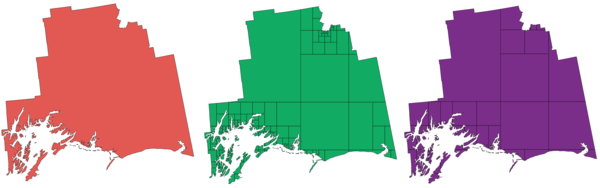Рис. 24.83 Left the input layer, middle maximum nodes value is 100 and right maximum value is 200

Примечание

Subdividing a geometry can generate geometry parts that may not be valid and may contain self-intersections.

См.также

Parameters¶

Label

Имя

Тип

Описание

Input layer

INPUT

[vector: any]

The input vector layer

Maximum nodes in parts

MAX_NODES

Default: 256

Maximum number of vertices each new geometry part is allowed to have. Fewer sub-parts for higher values.

Subdivided

OUTPUT

[same as input]

Default: [Create temporary layer]

Specify the output (subdivided) vector layer. One of:

• Create Temporary Layer (TEMPORARY_OUTPUT)

• Save to File…

• Save to Geopackage…

• Save to PostGIS Table…

The file encoding can also be changed here.

Outputs¶

Label

Имя

Тип

Описание

Subdivided

OUTPUT

[same as input]

Output vector layer

Python code¶

Algorithm ID: native:subdivide

import processing
processing.run("algorithm_id", {parameter_dictionary})

The algorithm id is displayed when you hover over the algorithm in the Processing Toolbox. The parameter dictionary provides the parameter NAMEs and values. See Изпользование алгоритмов геообработки в консоли for details on how to run processing algorithms from the Python console.

24.1.16.75. Swap X and Y coordinates¶

Switches the X and Y coordinate values in input geometries.

It can be used to repair geometries which have accidentally had their latitude and longitude values reversed.

См.также

Parameters¶

Label

Имя

Тип

Описание

Input layer

INPUT

[vector: any]

The input vector layer

Swapped

OUTPUT

[same as input]

Default: [Create temporary layer]

Specify the output vector layer. One of:

• Create Temporary Layer (TEMPORARY_OUTPUT)

• Save to File…

• Save to Geopackage…

• Save to PostGIS Table…

The file encoding can also be changed here.

Outputs¶

Label

Имя

Тип

Описание

Swapped

OUTPUT

[same as input]

Output (swapped) vector layer

Python code¶

Algorithm ID: native:swapxy

import processing
processing.run("algorithm_id", {parameter_dictionary})

The algorithm id is displayed when you hover over the algorithm in the Processing Toolbox. The parameter dictionary provides the parameter NAMEs and values. See Изпользование алгоритмов геообработки в консоли for details on how to run processing algorithms from the Python console.

24.1.16.76. Tapered buffers¶

Creates tapered buffer along line geometries, using a specified start and end buffer diameter.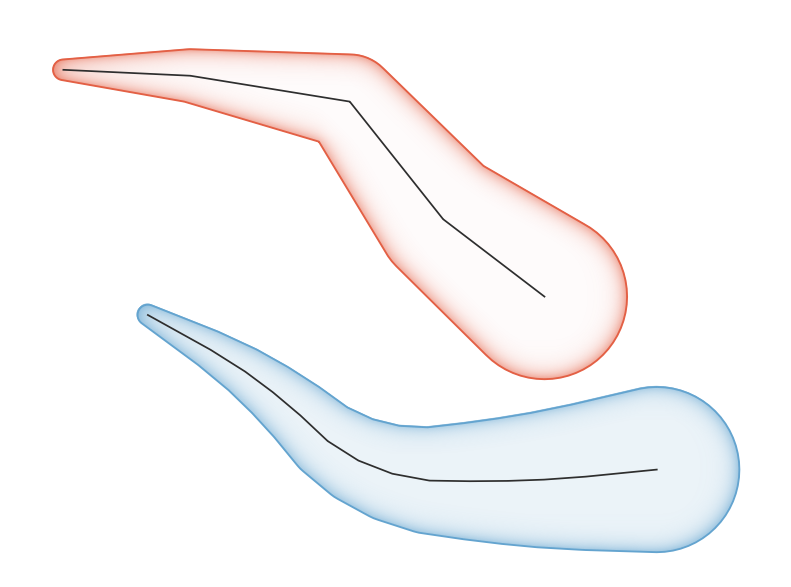Рис. 24.84 Tapered buffer example

Parameters¶

Label

Имя

Тип

Описание

Input layer

INPUT

[vector: line]

Input line vector layer

Start width

START_WIDTH

Default: 0.0

Represents the radius of the buffer applied at the start point of the line feature

End width

END_WIDTH

Default: 0.0

Represents the radius of the buffer applied at the end point of the line feature.

Segments

SEGMENTS

Default: 16

Controls the number of line segments to use to approximate a quarter circle when creating rounded offsets.

Buffered

OUTPUT

[vector: polygon]

Default: [Create temporary layer]

Specify the output (buffer) layer. One of:

• Create Temporary Layer (TEMPORARY_OUTPUT)

• Save to File…

• Save to Geopackage…

• Save to PostGIS Table…

The file encoding can also be changed here.

Outputs¶

Label

Имя

Тип

Описание

Buffered

OUTPUT

[vector: polygon]

Output (buffer) polygon layer

Python code¶

Algorithm ID: native:taperedbuffer

import processing
processing.run("algorithm_id", {parameter_dictionary})

The algorithm id is displayed when you hover over the algorithm in the Processing Toolbox. The parameter dictionary provides the parameter NAMEs and values. See Изпользование алгоритмов геообработки в консоли for details on how to run processing algorithms from the Python console.

24.1.16.77. Tessellate¶

Tessellates a polygon geometry layer, dividing the geometries into triangular components.

The output layer consists of multipolygon geometries for each input feature, with each multipolygon consisting of multiple triangle component polygons.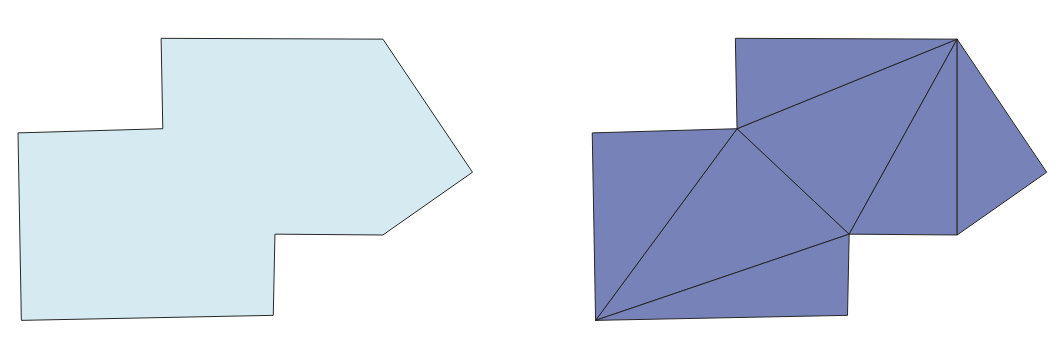Рис. 24.85 Tessellated polygon (right)

Parameters¶

Label

Имя

Тип

Описание

Input layer

INPUT

[vector: polygon]

Input polygon vector layer

Tesselated

OUTPUT

[vector: polygon]

Default: [Create temporary layer]

Specify the output layer. One of:

• Create Temporary Layer (TEMPORARY_OUTPUT)

• Save to File…

• Save to Geopackage…

• Save to PostGIS Table…

The file encoding can also be changed here.

Outputs¶

Label

Имя

Тип

Описание

Tesselated

OUTPUT

[vector: polygon]

Output multipolygonZ layer

Python code¶

Algorithm ID: 3d:tessellate

import processing
processing.run("algorithm_id", {parameter_dictionary})

The algorithm id is displayed when you hover over the algorithm in the Processing Toolbox. The parameter dictionary provides the parameter NAMEs and values. See Изпользование алгоритмов геообработки в консоли for details on how to run processing algorithms from the Python console.

24.1.16.78. Transect¶

Creates transects on vertices for (multi)linestring.

A transect is a line oriented from an angle (by default perpendicular) to the input polylines (at vertices).

Field(s) from feature(s) are returned in the transect with these new fields:

• TR_FID: ID of the original feature

• TR_ID: ID of the transect. Each transect have an unique ID

• TR_SEGMENT: ID of the segment of the linestring

• TR_ANGLE: Angle in degrees from the original line at the vertex

• TR_LENGTH: Total length of the transect returned

• TR_ORIENT: Side of the transect (only on the left or right of the line, or both side)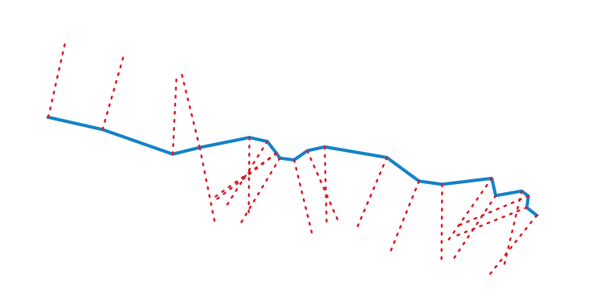Рис. 24.86 Dashed red lines represent the transect of the input line layer

Parameters¶

Label

Имя

Тип

Описание

Input layer

INPUT

[vector: line]

Input line vector layer

Length of the transect

LENGTH

Default: 5.0

Length in map unit of the transect

Angle in degrees from the original line at the vertices

ANGLE

Default: 90.0

Change the angle of the transect

Side to create the transect

SIDE

[enumeration]

Choose the side of the transect. Available options are:

• 0 — Left

• 1 — Right

• 2 — Both

Transect

OUTPUT

[vector: line]

Default: [Create temporary layer]

Specify the output line layer. One of:

• Create Temporary Layer (TEMPORARY_OUTPUT)

• Save to File…

• Save to Geopackage…

• Save to PostGIS Table…

The file encoding can also be changed here.

Outputs¶

Label

Имя

Тип

Описание

Transect

OUTPUT

[vector: line]

Output line layer

Python code¶

Algorithm ID: native:transect

import processing
processing.run("algorithm_id", {parameter_dictionary})

The algorithm id is displayed when you hover over the algorithm in the Processing Toolbox. The parameter dictionary provides the parameter NAMEs and values. See Изпользование алгоритмов геообработки в консоли for details on how to run processing algorithms from the Python console.

24.1.16.79. Translate¶

Moves the geometries within a layer, by offsetting with a predefined X and Y displacement.

Z and M values present in the geometry can also be translated.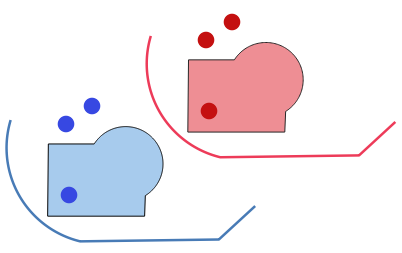Рис. 24.87 Dashed lines represent the translated geometry of the input layer

Parameters¶

Label

Имя

Тип

Описание

Input layer

INPUT

[vector: any]

Input vector layer

Offset distance (x-axis)

DELTA_X

Default: 0.0

Displacement to apply on the X axis

Offset distance (y-axis)

DELTA_Y

Default: 0.0

Displacement to apply on the Y axis

Offset distance (z-axis)

DELTA_Z

Default: 0.0

Displacement to apply on the Z axis

Offset distance (m values)

DELTA_M

Default: 0.0

Displacement to apply on the M axis

Translated

OUTPUT

[same as input]

Default: [Create temporary layer]

Specify the output vector layer. One of:

• Create Temporary Layer (TEMPORARY_OUTPUT)

• Save to File…

• Save to Geopackage…

• Save to PostGIS Table…

The file encoding can also be changed here.

Outputs¶

Label

Имя

Тип

Описание

Translated

OUTPUT

[same as input]

Output vector layer

Python code¶

Algorithm ID: native:translategeometry

import processing
processing.run("algorithm_id", {parameter_dictionary})

The algorithm id is displayed when you hover over the algorithm in the Processing Toolbox. The parameter dictionary provides the parameter NAMEs and values. See Изпользование алгоритмов геообработки в консоли for details on how to run processing algorithms from the Python console.

24.1.16.80. Variable distance buffer¶

Computes a buffer area for all the features in an input layer.

The size of the buffer for a given feature is defined by an attribute, so it allows different features to have different buffer sizes.

Примечание

This algorithm is only available from the Graphical modeler.

См.также

Buffer

Parameters¶

Label

Имя

Тип

Описание

Input layer

INPUT

[vector: any]

Input vector layer

Distance field

DISTANCE

[tablefield: numeric]

Attribute for the distance radius of the buffer

Segments

SEGMENTS

[number]

Default: 5

Controls the number of line segments to use to approximate a quarter circle when creating rounded offsets.

Dissolve result

DISSOLVE

[boolean]

Default: False

Choose to dissolve the final buffer, resulting in a single feature covering all input features.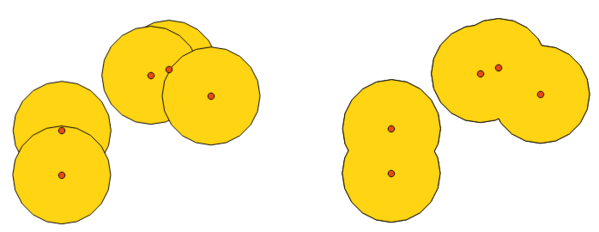Рис. 24.88 Normal and dissolved buffer

End cap style

END_CAP_STYLE

[enumeration]

Controls how line endings are handled in the buffer.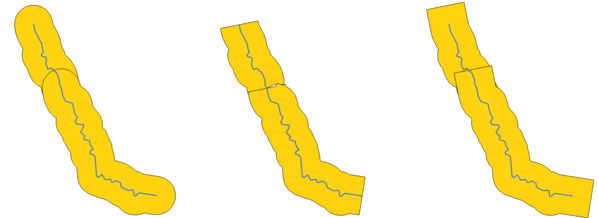Рис. 24.89 Round, flat and square cap styles

Join style

JOIN_STYLE

[enumeration]

Specifies whether round, miter or beveled joins should be used when offsetting corners in a line.

Miter limit

MITER_LIMIT

[number]

Default: 2.0

Only applicable for mitered join styles, and controls the maximum distance from the offset curve to use when creating a mitered join.

Outputs¶

Label

Имя

Тип

Описание

Buffer

OUTPUT

[vector: polygon]

Buffer polygon vector layer.

Python code¶

Algorithm ID: qgis:variabledistancebuffer

import processing
processing.run("algorithm_id", {parameter_dictionary})

The algorithm id is displayed when you hover over the algorithm in the Processing Toolbox. The parameter dictionary provides the parameter NAMEs and values. See Изпользование алгоритмов геообработки в консоли for details on how to run processing algorithms from the Python console.

24.1.16.81. Variable width buffer (by M value)¶

Creates variable width buffers along lines, using the M value of the line geometries as the diameter of the buffer at each vertex.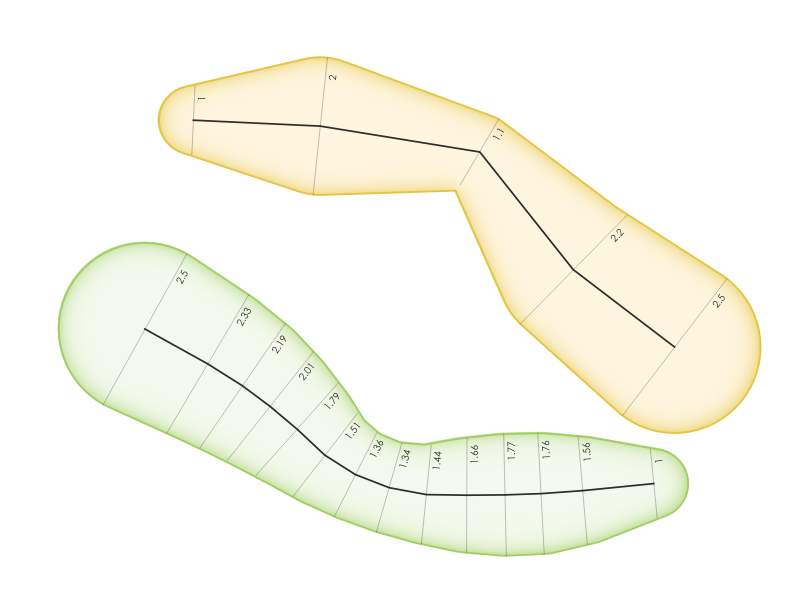Рис. 24.90 Variable buffer example

См.также

Parameters¶

Label

Имя

Тип

Описание

Input layer

INPUT

[vector: line]

Input line vector layer

Segments

SEGMENTS

Default: 16

Number of the buffer segments per quarter circle. It can be a unique value (same value for all the features), or it can be taken from features data (the value can depend on feature attributes).

Buffered

OUTPUT

[vector: polygon]

Default: [Create temporary layer]

Specify the output (buffer) layer. One of:

• Create Temporary Layer (TEMPORARY_OUTPUT)

• Save to File…

• Save to Geopackage…

• Save to PostGIS Table…

The file encoding can also be changed here.

Outputs¶

Label

Имя

Тип

Описание

Buffered

OUTPUT

[vector: polygon]

Variable buffer polygon layer

Python code¶

Algorithm ID: native:bufferbym

import processing
processing.run("algorithm_id", {parameter_dictionary})

The algorithm id is displayed when you hover over the algorithm in the Processing Toolbox. The parameter dictionary provides the parameter NAMEs and values. See Изпользование алгоритмов геообработки в консоли for details on how to run processing algorithms from the Python console.

24.1.16.82. Voronoi polygons¶

Takes a point layer and generates a polygon layer containing the Voronoi polygons (known also as Thiessen polygons) corresponding to those input points.

Any location within a Voronoi polygon is closer to the associated point than to any other point.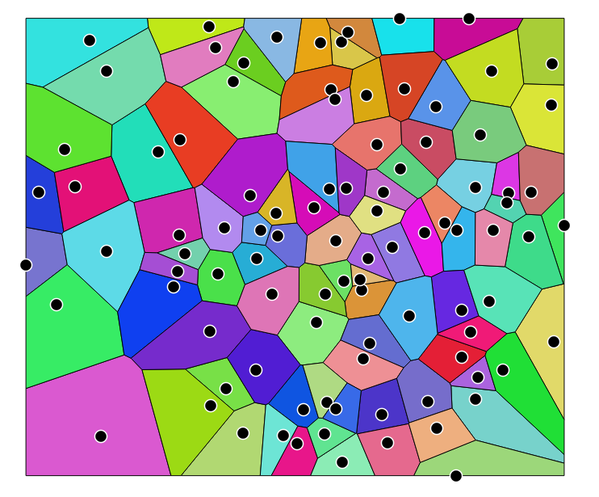Рис. 24.91 Voronoi polygons

Default menu: Vector ► Geometry Tools

Parameters¶

Label

Имя

Тип

Описание

Input layer

INPUT

[vector: point]

Input point vector layer

Buffer region (% of extent)

BUFFER

[number]

Default: 0.0

The extent of the output layer will be this much bigger than the extent of the input layer

Voronoi polygons

OUTPUT

[vector: polygon]

Default: [Create temporary layer]

Specify the output layer (with the Voronoi polygons). One of:

• Create Temporary Layer (TEMPORARY_OUTPUT)

• Save to File…

• Save to Geopackage…

• Save to PostGIS Table…

The file encoding can also be changed here.

Outputs¶

Label

Имя

Тип

Описание

Voronoi polygons

OUTPUT

[vector: polygon]

Voronoi polygons of the input point vector layer

Python code¶

Algorithm ID: qgis:voronoipolygons

import processing
processing.run("algorithm_id", {parameter_dictionary})

The algorithm id is displayed when you hover over the algorithm in the Processing Toolbox. The parameter dictionary provides the parameter NAMEs and values. See Изпользование алгоритмов геообработки в консоли for details on how to run processing algorithms from the Python console.